Скачать презентацию Return Risk and Rates of Return Risk

071cb1ab999a65c27dd6c56cb4656cd4.ppt

• Количество слайдов: 89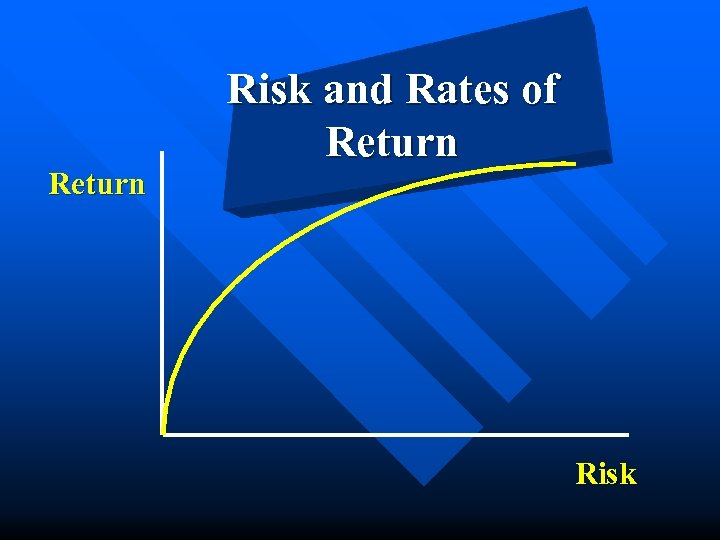Return Risk and Rates of Return Risk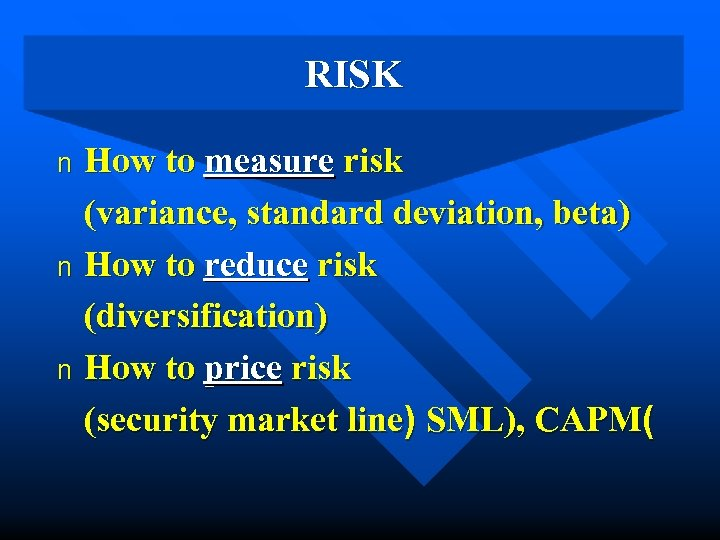RISK How to measure risk (variance, standard deviation, beta) n How to reduce risk (diversification) n How to price risk (security market line) SML), CAPM( n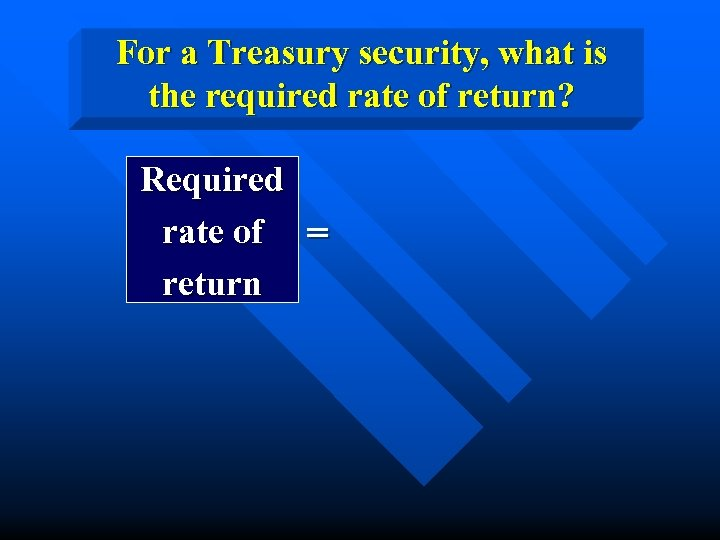For a Treasury security, what is the required rate of return? Required rate of = return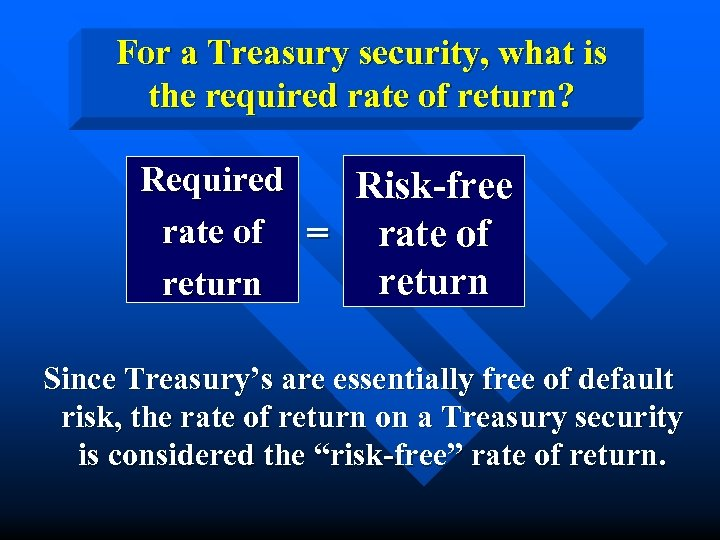For a Treasury security, what is the required rate of return? Required Risk-free rate of = rate of return Since Treasury’s are essentially free of default risk, the rate of return on a Treasury security is considered the “risk-free” rate of return.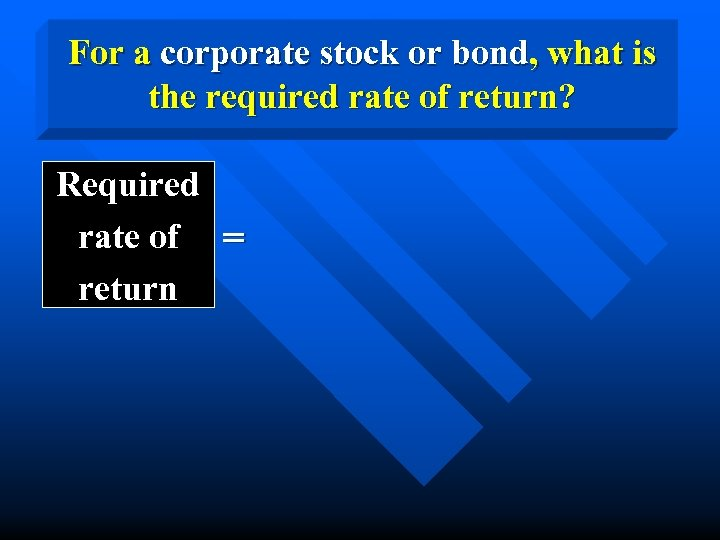For a corporate stock or bond, what is the required rate of return? Required rate of = return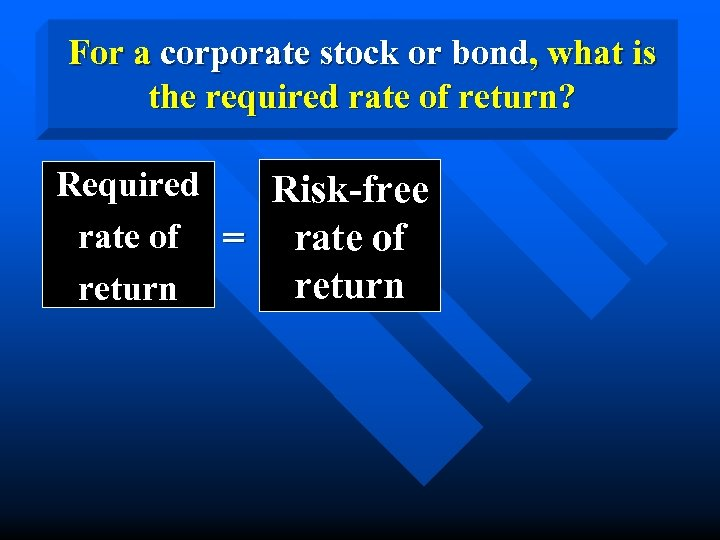For a corporate stock or bond, what is the required rate of return? Required Risk-free rate of = rate of return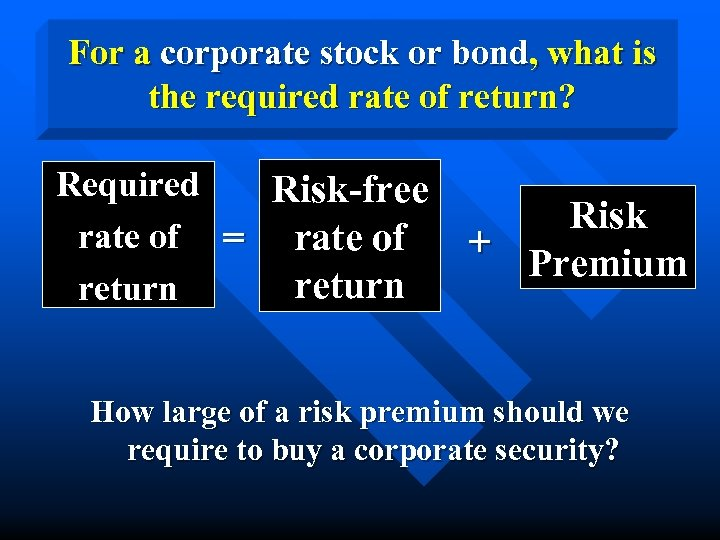For a corporate stock or bond, what is the required rate of return? Required Risk-free rate of = rate of return Risk + Premium How large of a risk premium should we require to buy a corporate security?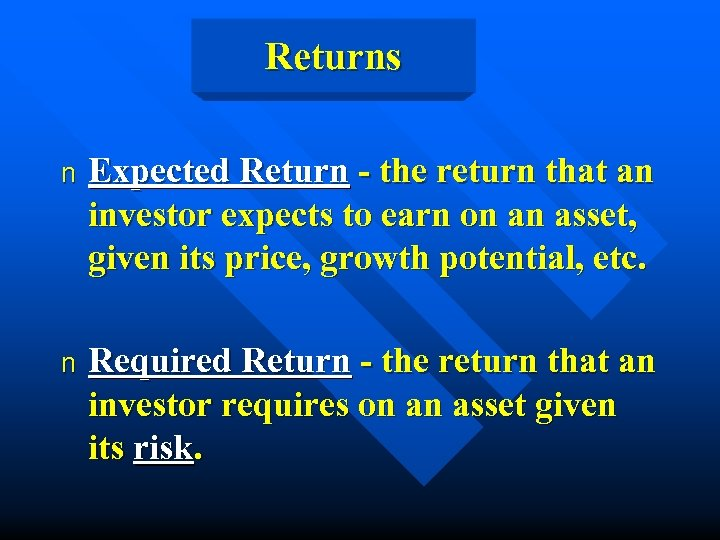Returns n Expected Return - the return that an investor expects to earn on an asset, given its price, growth potential, etc. n Required Return - the return that an investor requires on an asset given its risk.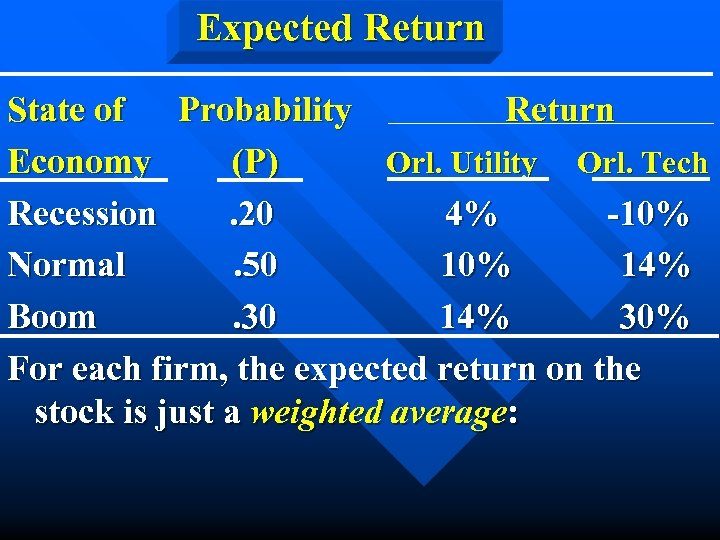Expected Return State of Probability Return Economy (P) Orl. Utility Orl. Tech Recession. 20 4% -10% Normal. 50 10% 14% Boom. 30 14% 30% For each firm, the expected return on the stock is just a weighted average: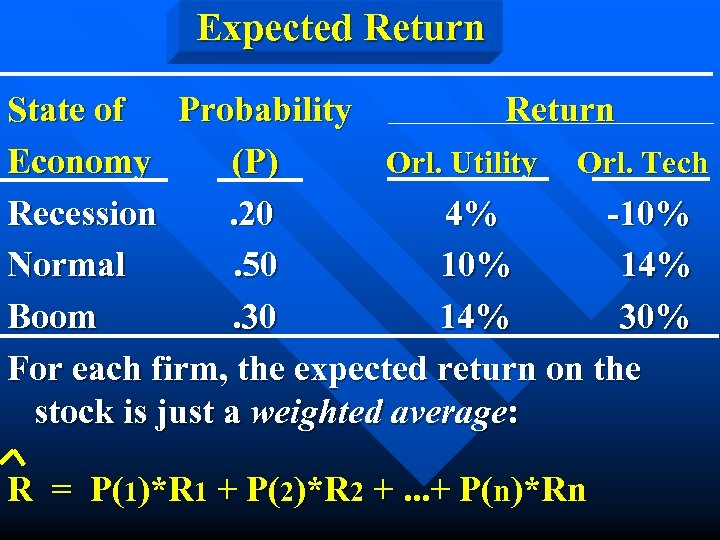Expected Return State of Probability Return Economy (P) Orl. Utility Orl. Tech Recession. 20 4% -10% Normal. 50 10% 14% Boom. 30 14% 30% For each firm, the expected return on the stock is just a weighted average: R = P(1)*R 1 + P(2)*R 2 +. . . + P(n)*Rn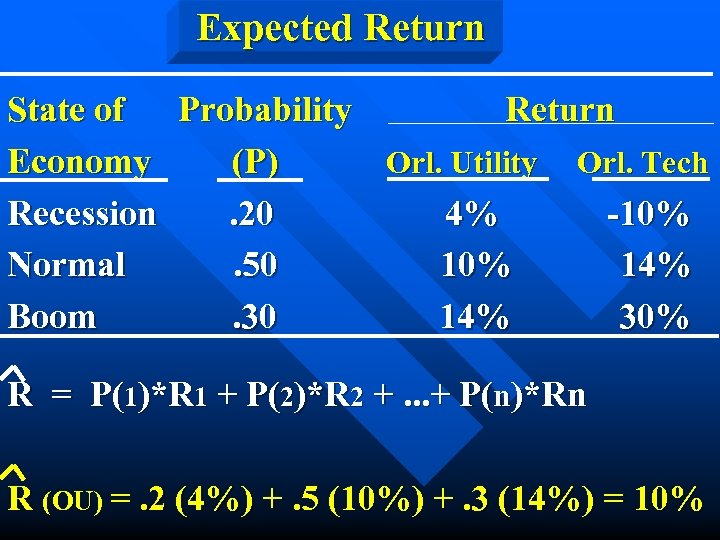Expected Return State of Probability Return Economy (P) Orl. Utility Orl. Tech Recession. 20 4% -10% Normal. 50 10% 14% Boom. 30 14% 30% R = P(1)*R 1 + P(2)*R 2 +. . . + P(n)*Rn R (OU) =. 2 (4%) +. 5 (10%) +. 3 (14%) = 10%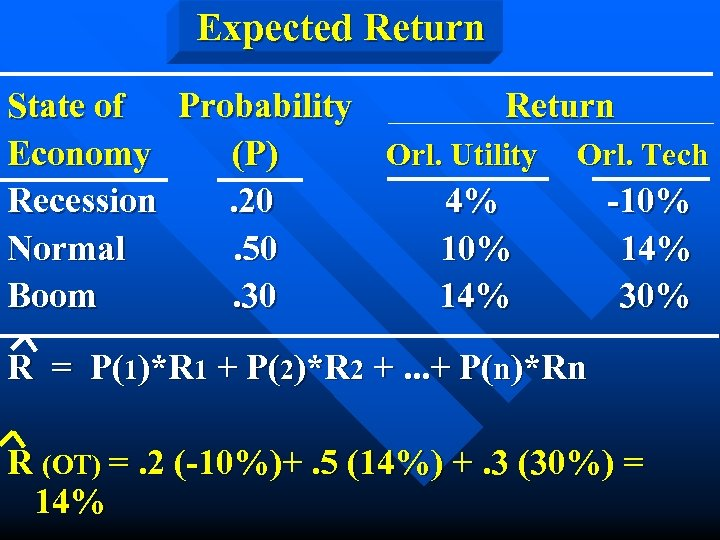Expected Return State of Probability Return Economy (P) Orl. Utility Orl. Tech Recession. 20 4% -10% Normal. 50 10% 14% Boom. 30 14% 30% R = P(1)*R 1 + P(2)*R 2 +. . . + P(n)*Rn R (OT) =. 2 (-10%)+. 5 (14%) +. 3 (30%) = 14%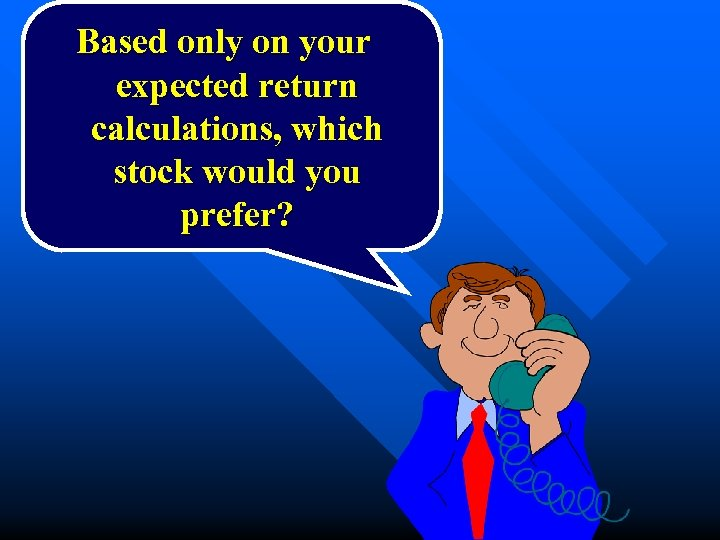Based only on your expected return calculations, which stock would you prefer?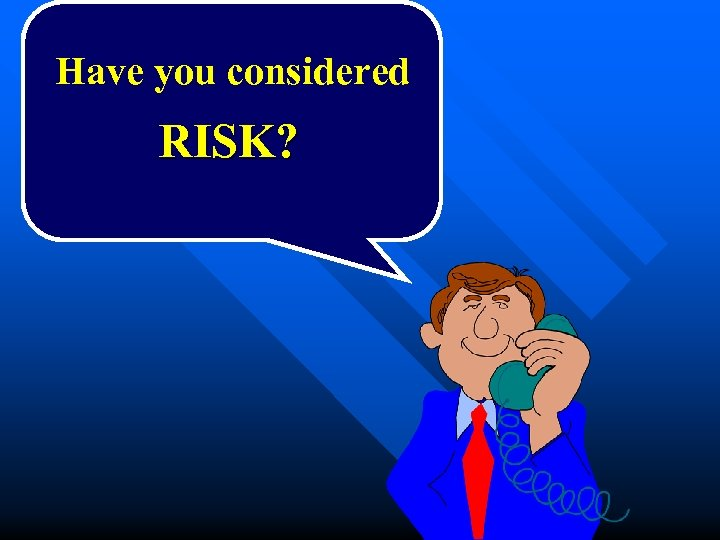Have you considered RISK?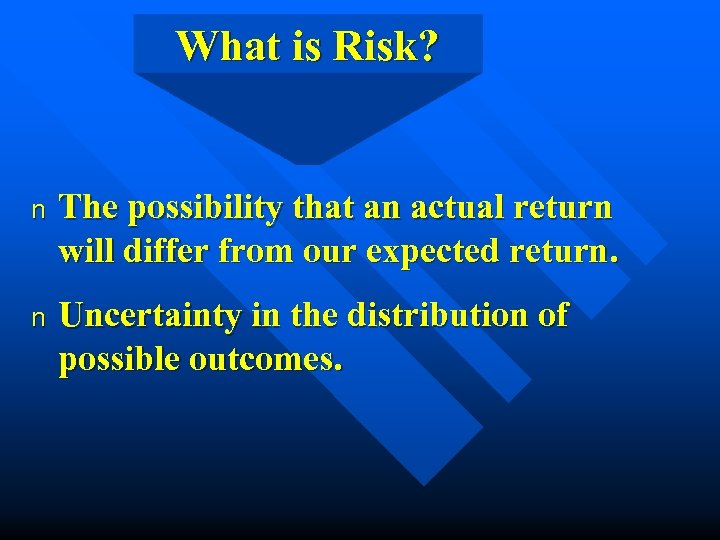What is Risk? n The possibility that an actual return will differ from our expected return. n Uncertainty in the distribution of possible outcomes.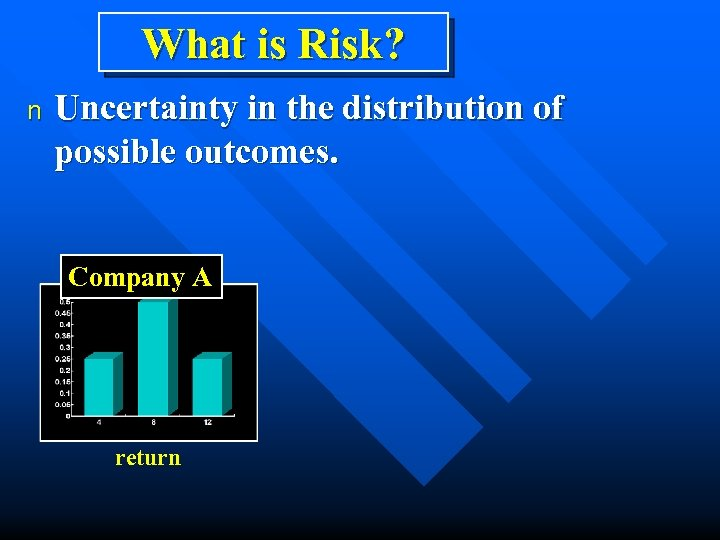What is Risk? n Uncertainty in the distribution of possible outcomes. Company A return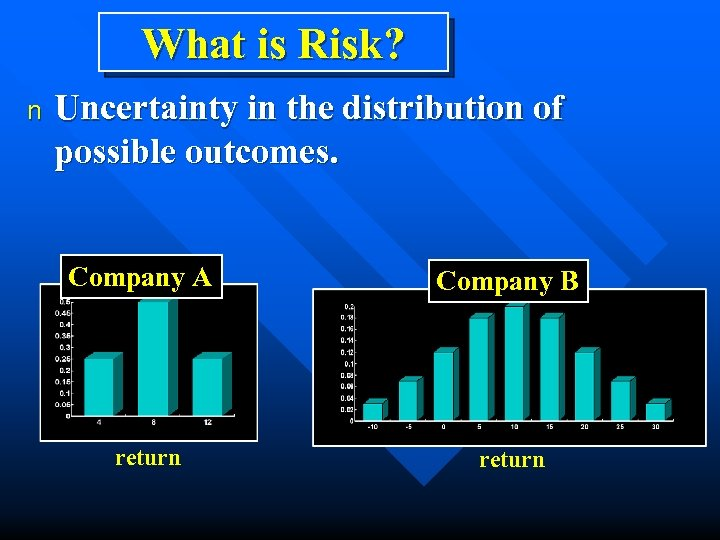What is Risk? n Uncertainty in the distribution of possible outcomes. Company A return Company B return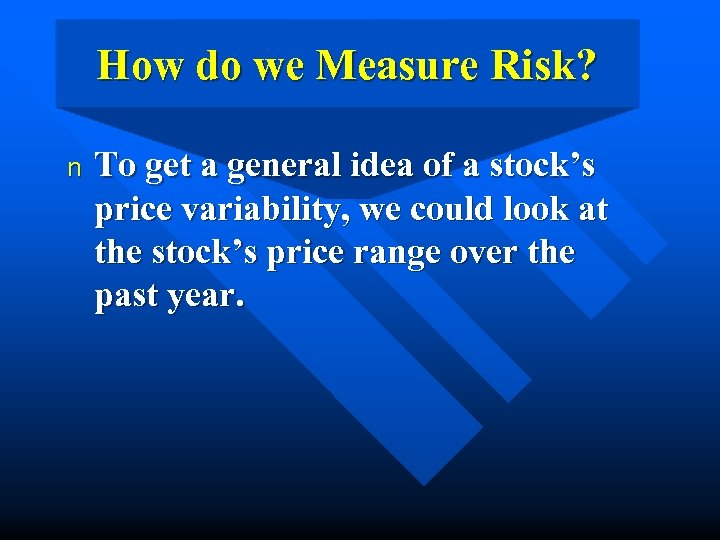How do we Measure Risk? n To get a general idea of a stock’s price variability, we could look at the stock’s price range over the past year.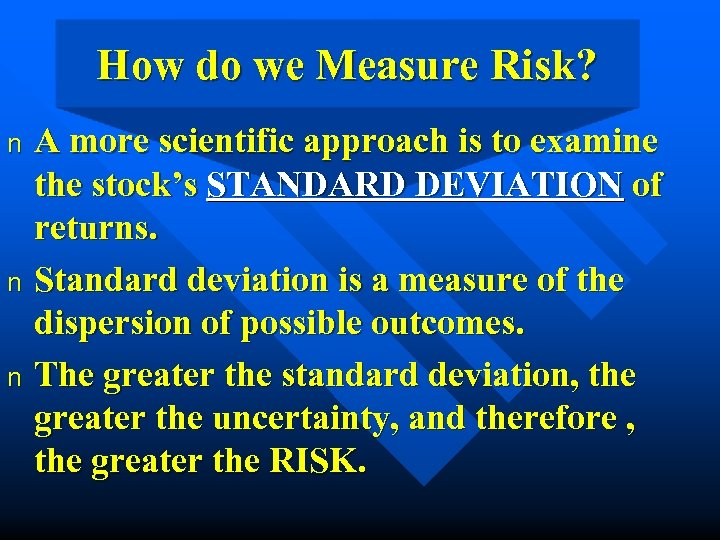How do we Measure Risk? A more scientific approach is to examine the stock’s STANDARD DEVIATION of returns. n Standard deviation is a measure of the dispersion of possible outcomes. n The greater the standard deviation, the greater the uncertainty, and therefore , the greater the RISK. n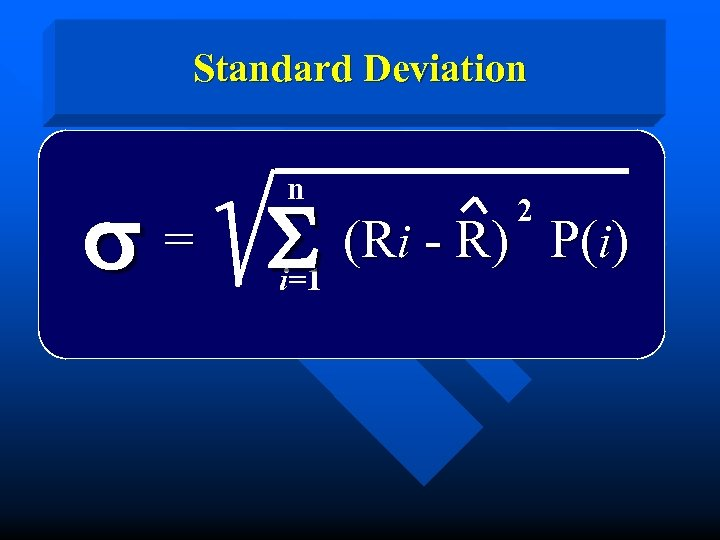Standard Deviation n s = S (Ri - R) i=1 2 P(i)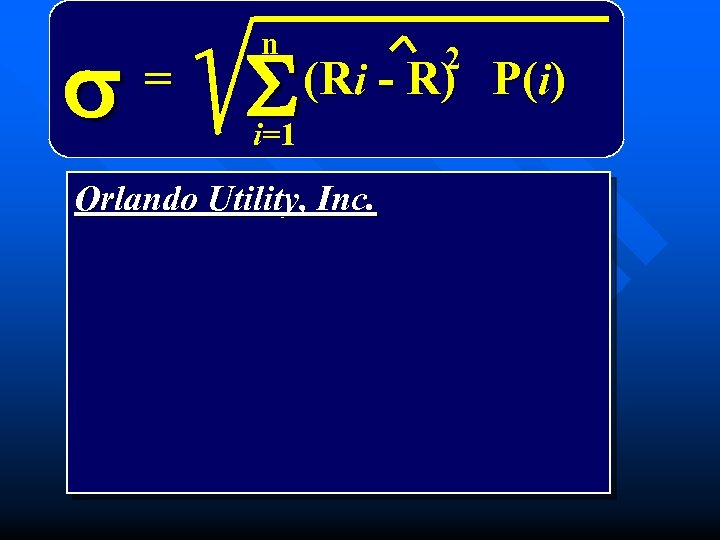s = n S 2 (Ri - R) P(i) i=1 Orlando Utility, Inc.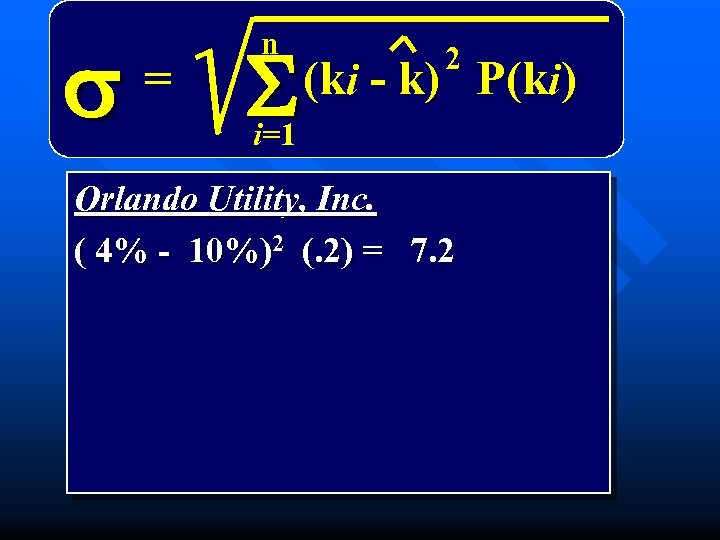s = n n S 2 (ki - k) P(ki) i=1 Orlando Utility, Inc. ( 4% - 10%)2 (. 2) = 7. 2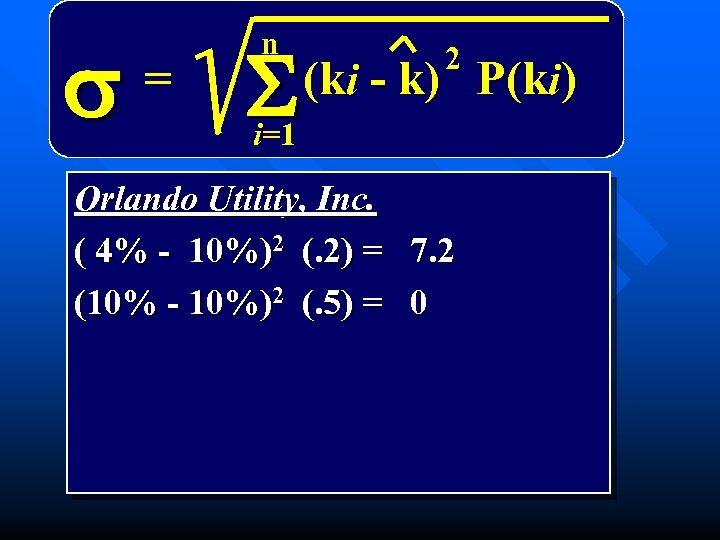s = n S 2 (ki - k) P(ki) i=1 Orlando Utility, Inc. ( 4% - 10%)2 (. 2) = 7. 2 (10% - 10%)2 (. 5) = 0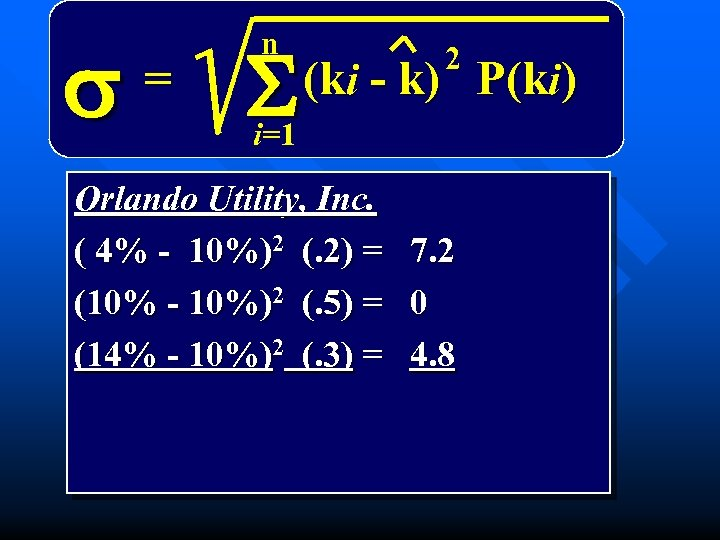s = n S 2 (ki - k) P(ki) i=1 Orlando Utility, Inc. ( 4% - 10%)2 (. 2) = 7. 2 (10% - 10%)2 (. 5) = 0 (14% - 10%)2 (. 3) = 4. 8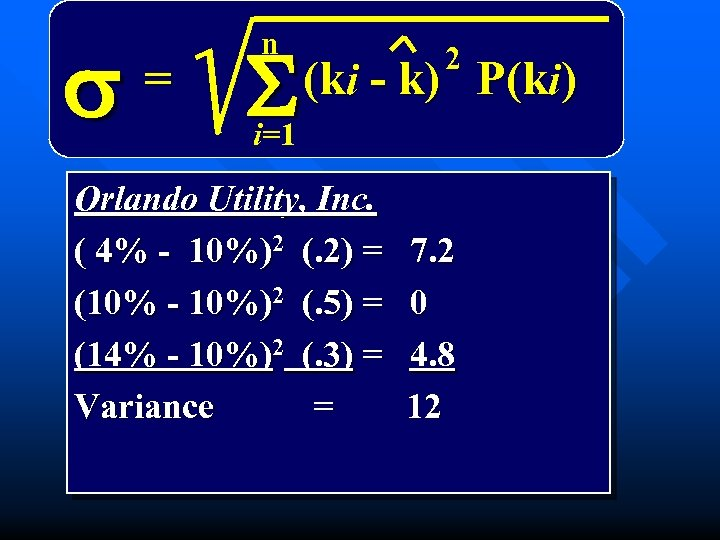s = n S 2 (ki - k) P(ki) i=1 Orlando Utility, Inc. ( 4% - 10%)2 (. 2) = (10% - 10%)2 (. 5) = (14% - 10%)2 (. 3) = Variance = 7. 2 0 4. 8 12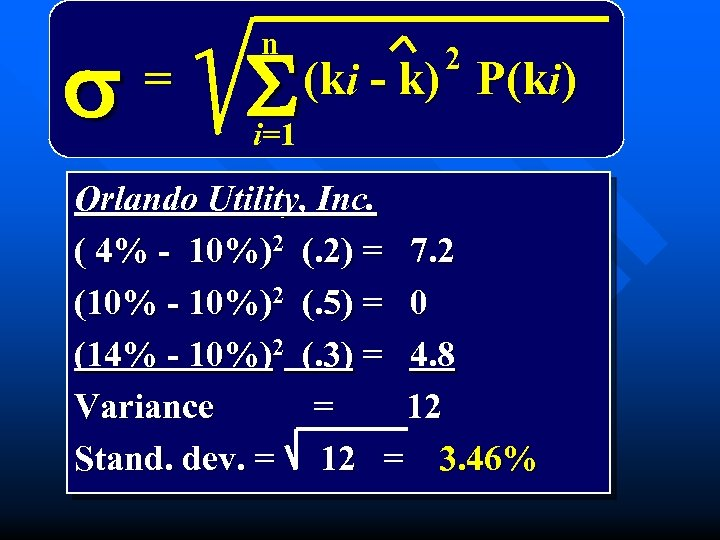s = n S 2 (ki - k) P(ki) i=1 Orlando Utility, Inc. ( 4% - 10%)2 (. 2) = 7. 2 (10% - 10%)2 (. 5) = 0 (14% - 10%)2 (. 3) = 4. 8 Variance = 12 Stand. dev. = 12 = 3. 46%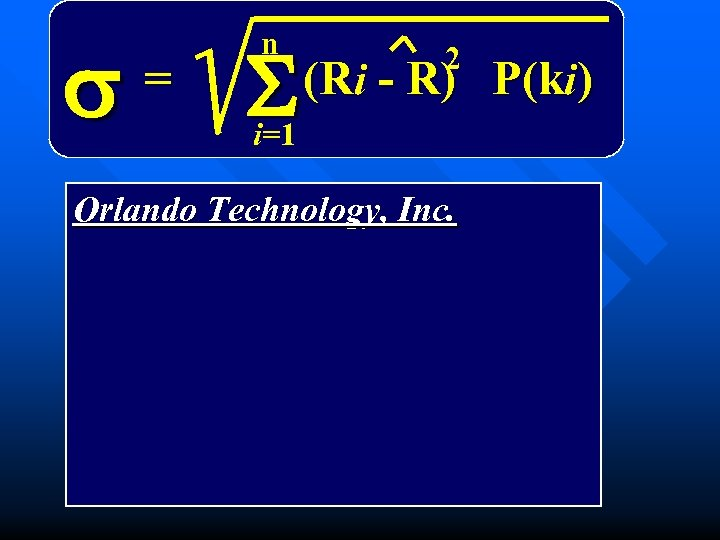s = n S 2 (Ri - R) P(ki) i=1 Orlando Technology, Inc.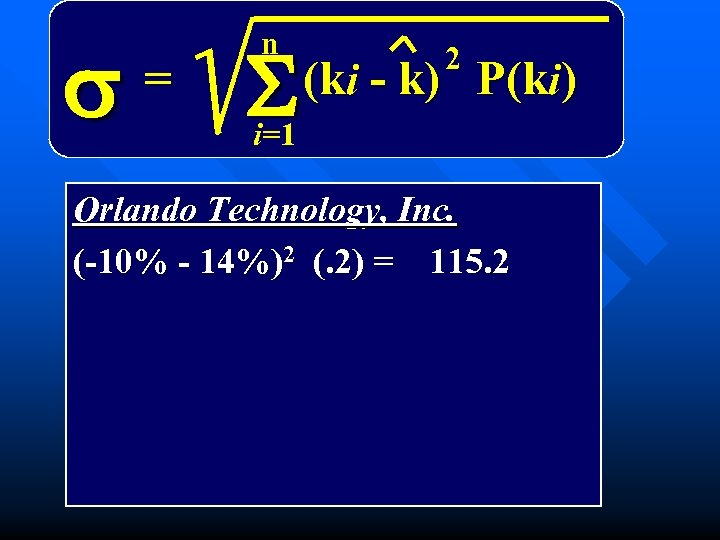s = n S 2 (ki - k) P(ki) i=1 Orlando Technology, Inc. (-10% - 14%)2 (. 2) = 115. 2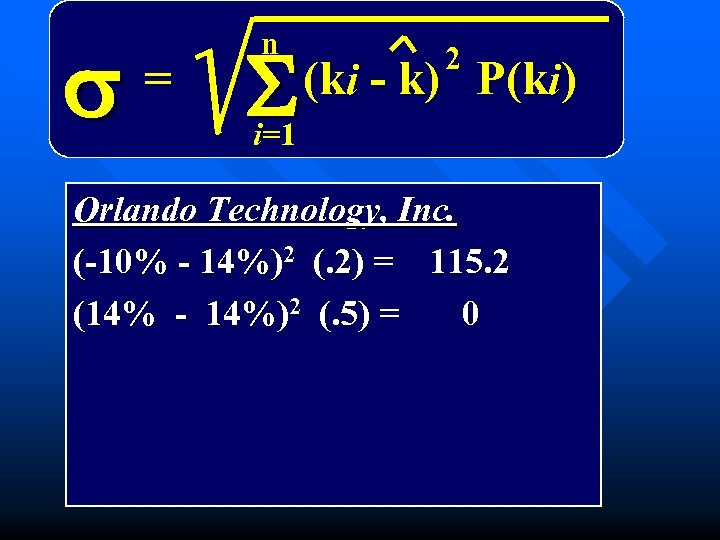s = n S 2 (ki - k) P(ki) i=1 Orlando Technology, Inc. (-10% - 14%)2 (. 2) = 115. 2 (14% - 14%)2 (. 5) = 0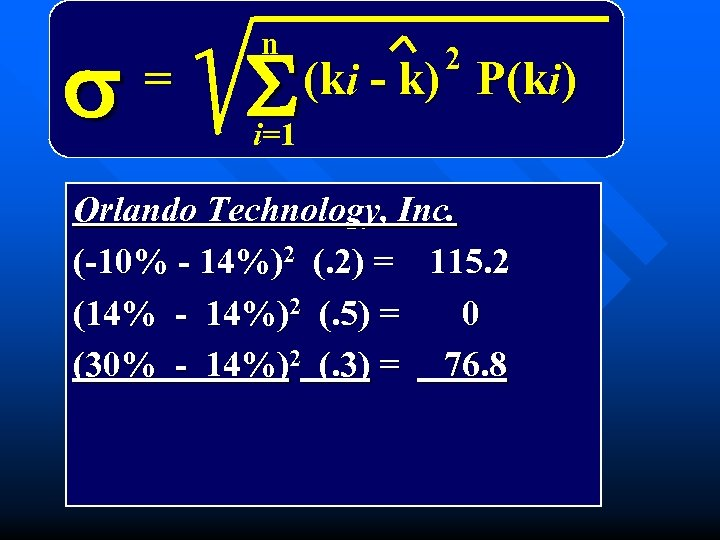s = n S 2 (ki - k) P(ki) i=1 Orlando Technology, Inc. (-10% - 14%)2 (. 2) = 115. 2 (14% - 14%)2 (. 5) = 0 (30% - 14%)2 (. 3) = 76. 8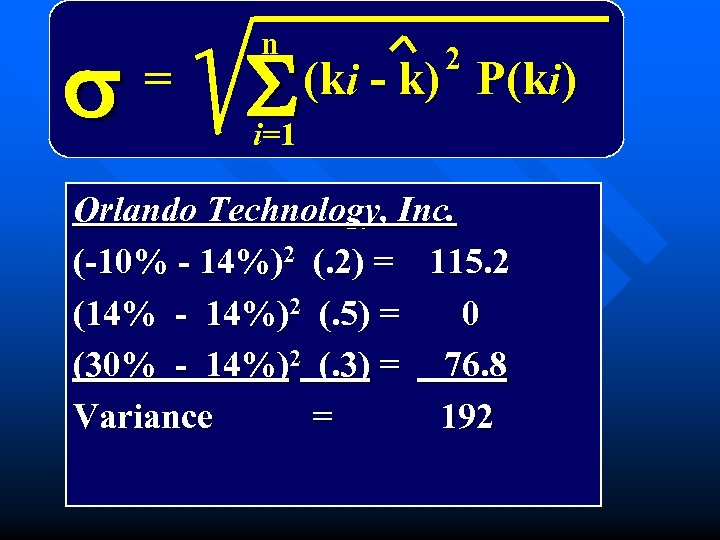s = n S 2 (ki - k) P(ki) i=1 Orlando Technology, Inc. (-10% - 14%)2 (. 2) = 115. 2 (14% - 14%)2 (. 5) = 0 (30% - 14%)2 (. 3) = 76. 8 Variance = 192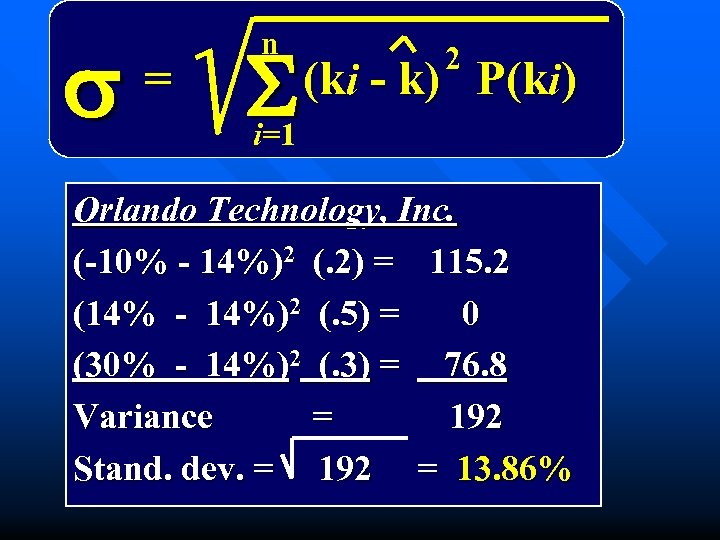s = n S 2 (ki - k) P(ki) i=1 Orlando Technology, Inc. (-10% - 14%)2 (. 2) = 115. 2 (14% - 14%)2 (. 5) = 0 (30% - 14%)2 (. 3) = 76. 8 Variance = 192 Stand. dev. = 192 = 13. 86%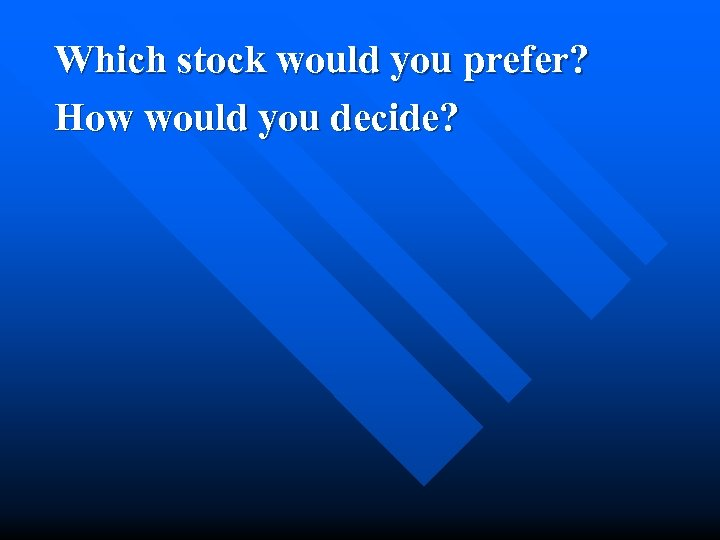Which stock would you prefer? How would you decide?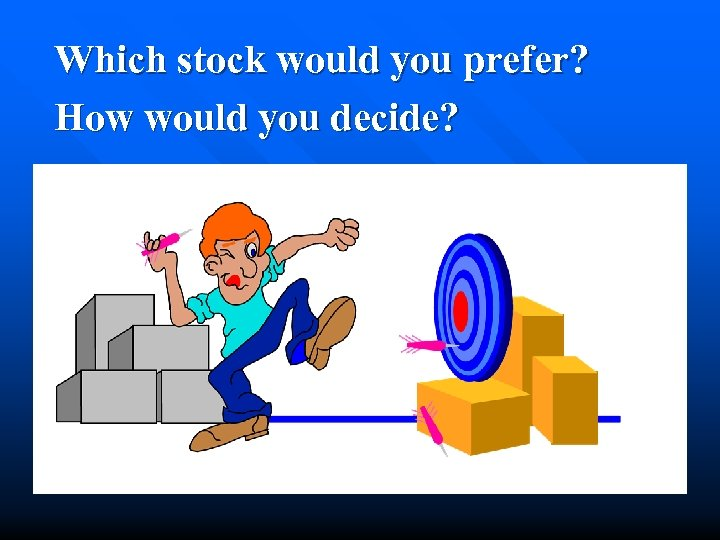Which stock would you prefer? How would you decide?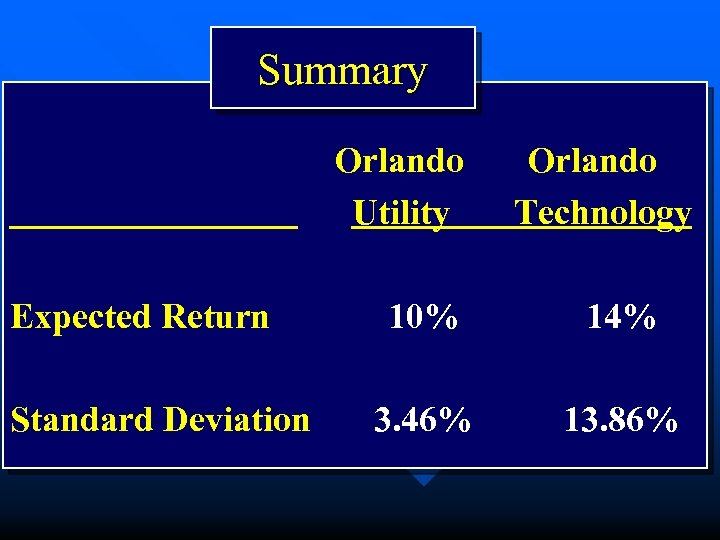Summary Orlando Utility Expected Return Standard Deviation Orlando Technology 10% 14% 3. 46% 13. 86%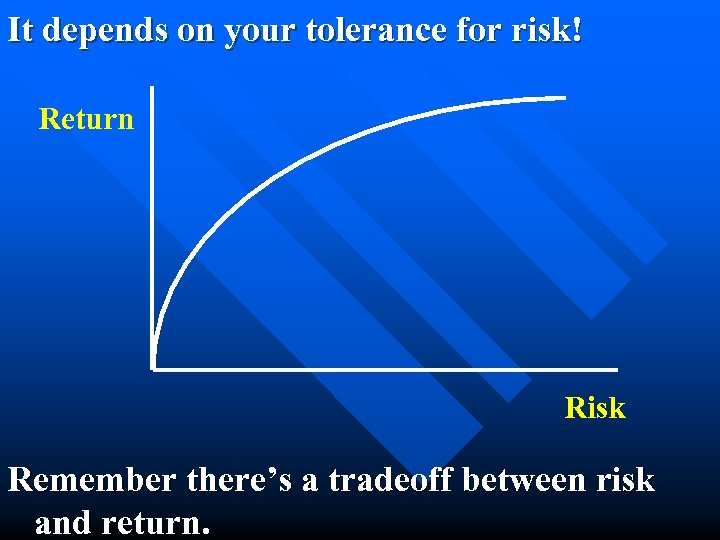It depends on your tolerance for risk! Return Risk Remember there’s a tradeoff between risk and return.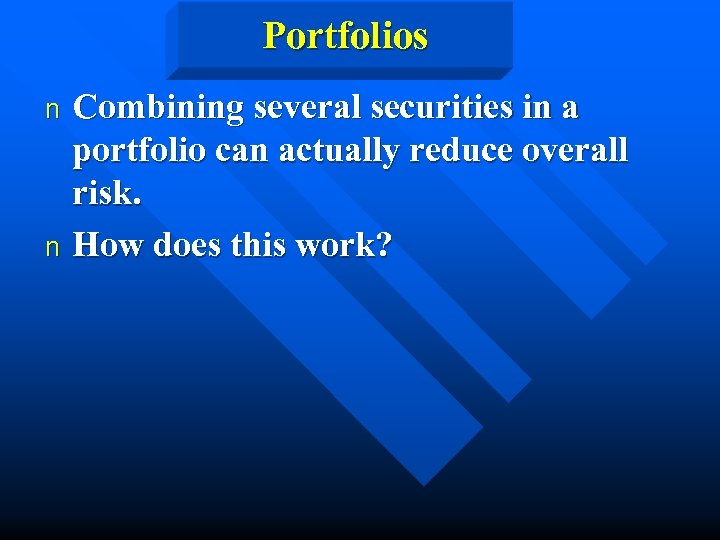Portfolios Combining several securities in a portfolio can actually reduce overall risk. n How does this work? n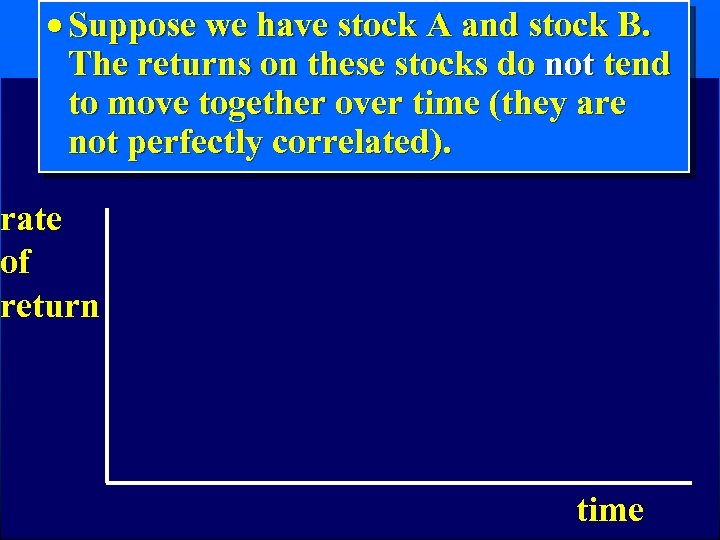· Suppose we have stock A and stock B. The returns on these stocks do not tend to move together over time (they are not perfectly correlated). rate of return time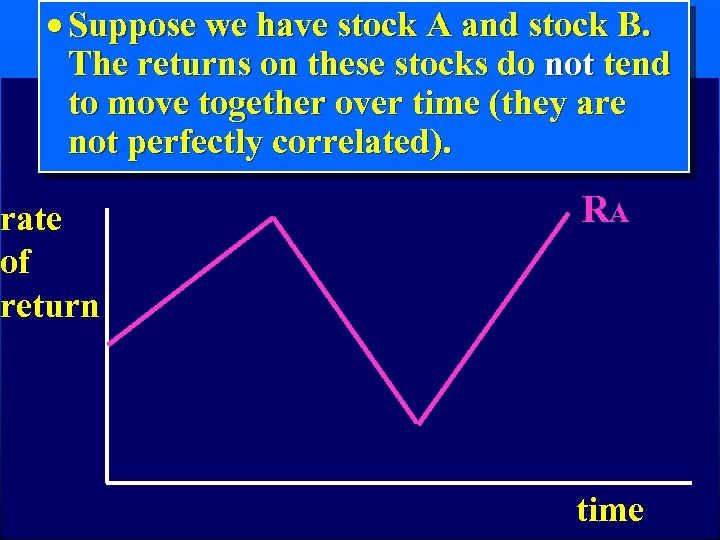· Suppose we have stock A and stock B. The returns on these stocks do not tend to move together over time (they are not perfectly correlated). rate of return RA time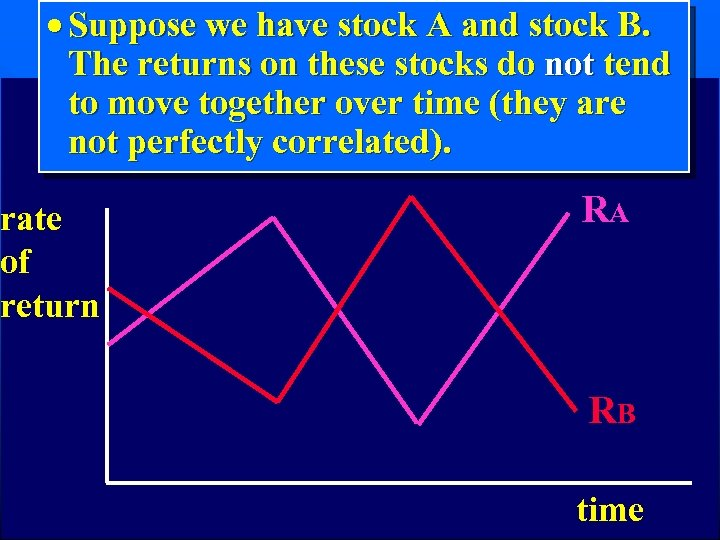· Suppose we have stock A and stock B. The returns on these stocks do not tend to move together over time (they are not perfectly correlated). rate of return RA RB time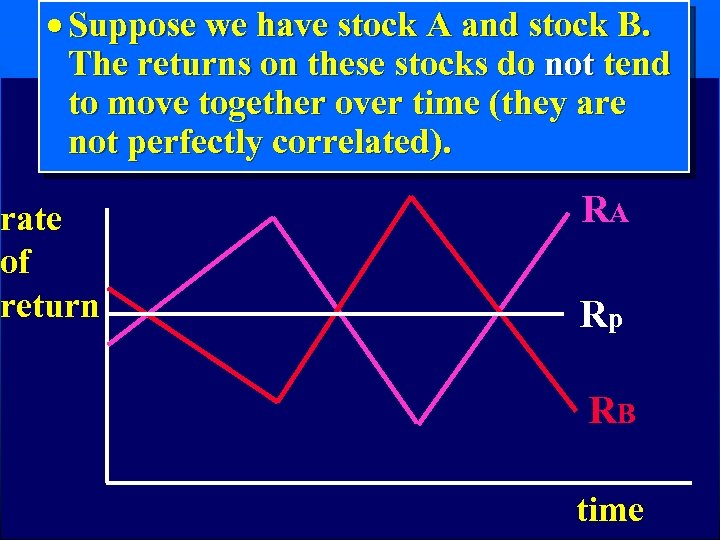· Suppose we have stock A and stock B. The returns on these stocks do not tend to move together over time (they are not perfectly correlated). rate of return RA Rp RB time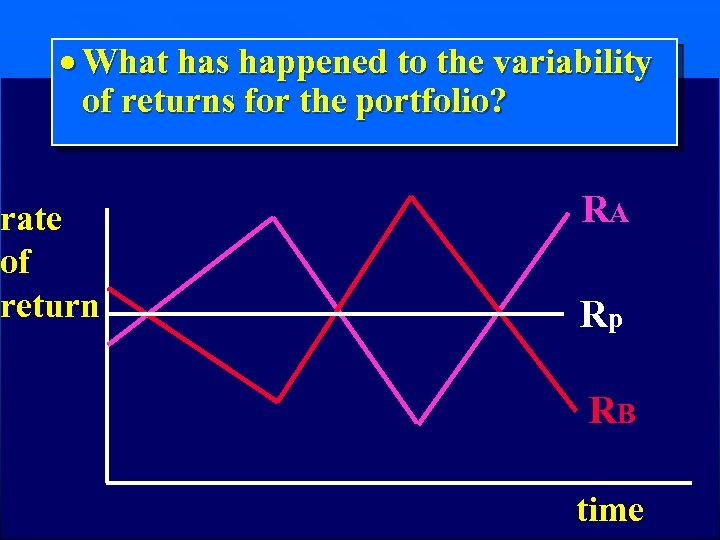· What has happened to the variability of returns for the portfolio? rate of return RA Rp RB time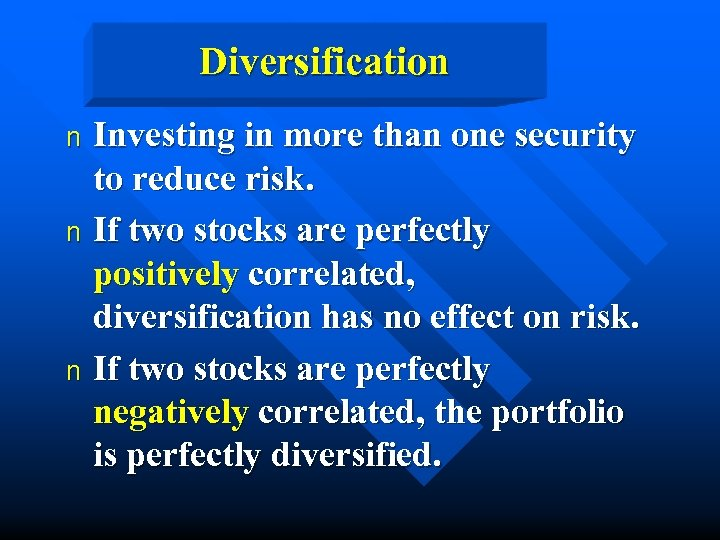Diversification Investing in more than one security to reduce risk. n If two stocks are perfectly positively correlated, diversification has no effect on risk. n If two stocks are perfectly negatively correlated, the portfolio is perfectly diversified. nIf you owned a share of every stock traded on the NYSE and NASDAQ, would you be diversified? YES! n Would you have eliminated all of your risk? NO! Common stock portfolios still have risk. n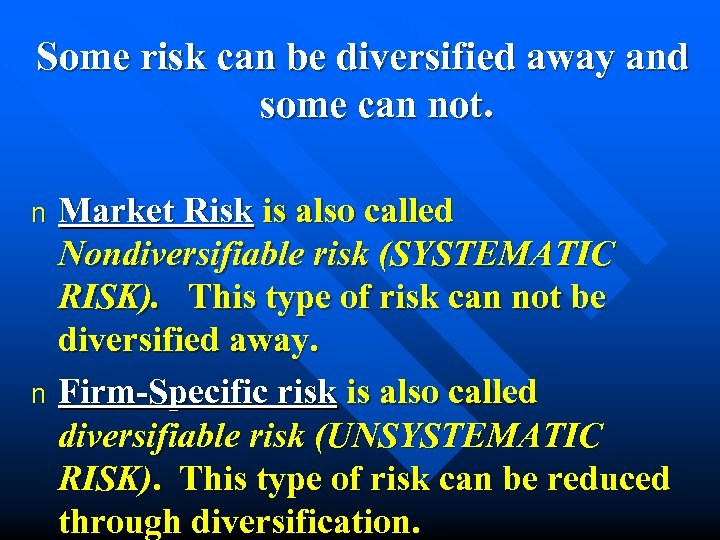Some risk can be diversified away and some can not. Market Risk is also called Nondiversifiable risk (SYSTEMATIC RISK). This type of risk can not be diversified away. n Firm-Specific risk is also called diversifiable risk (UNSYSTEMATIC RISK). This type of risk can be reduced through diversification. n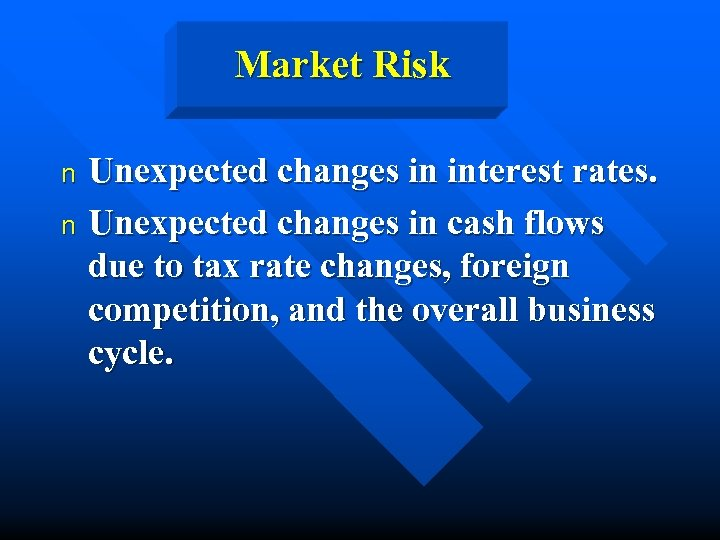Market Risk Unexpected changes in interest rates. n Unexpected changes in cash flows due to tax rate changes, foreign competition, and the overall business cycle. n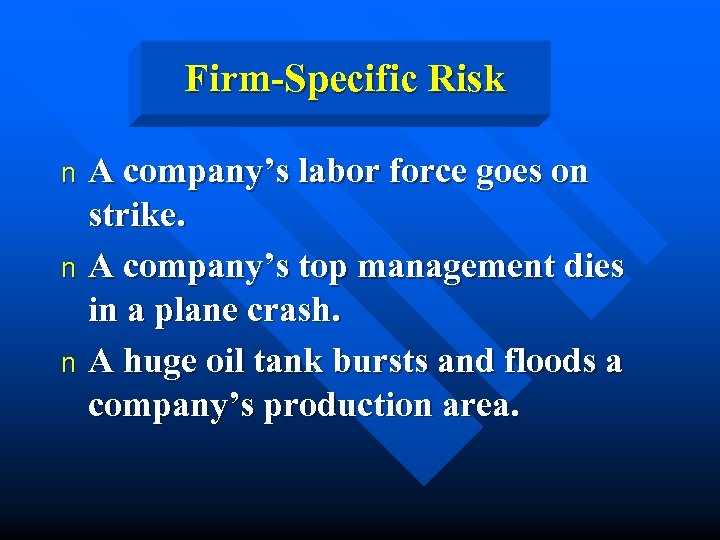Firm-Specific Risk A company’s labor force goes on strike. n A company’s top management dies in a plane crash. n A huge oil tank bursts and floods a company’s production area. n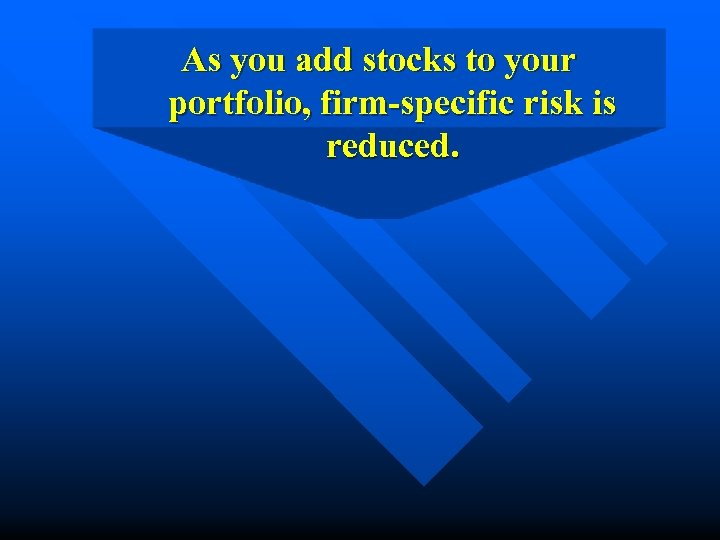As you add stocks to your portfolio, firm-specific risk is reduced.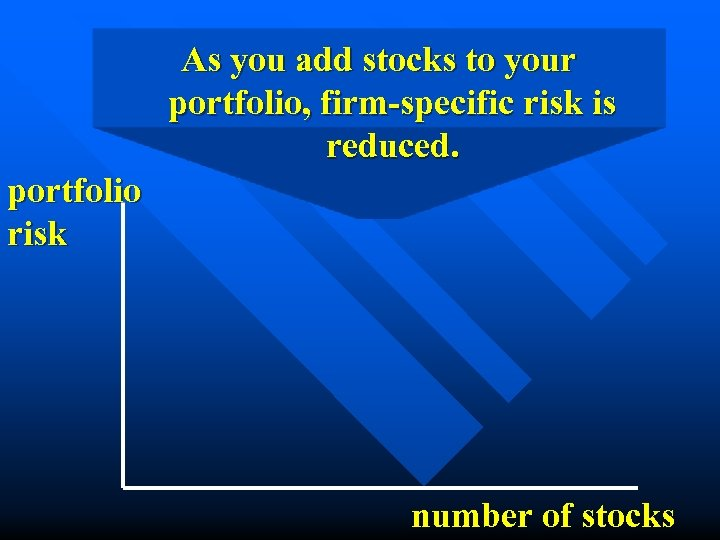As you add stocks to your portfolio, firm-specific risk is reduced. portfolio risk number of stocks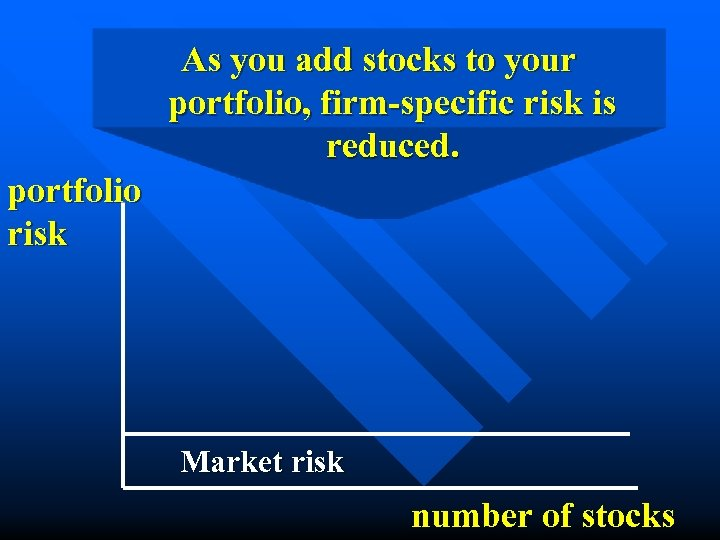As you add stocks to your portfolio, firm-specific risk is reduced. portfolio risk Market risk number of stocks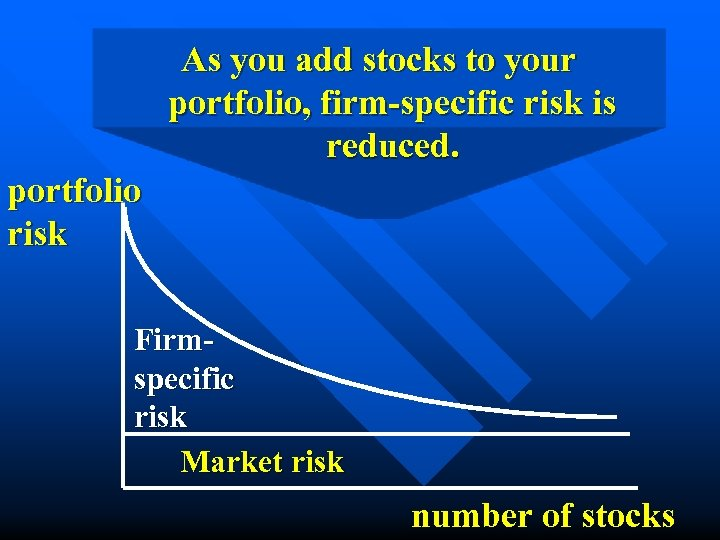As you add stocks to your portfolio, firm-specific risk is reduced. portfolio risk Firmspecific risk Market risk number of stocks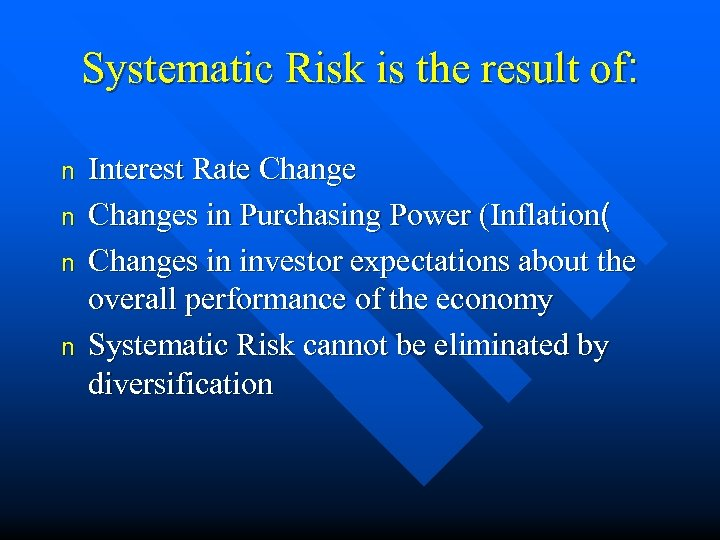Systematic Risk is the result of: n n Interest Rate Changes in Purchasing Power (Inflation( Changes in investor expectations about the overall performance of the economy Systematic Risk cannot be eliminated by diversification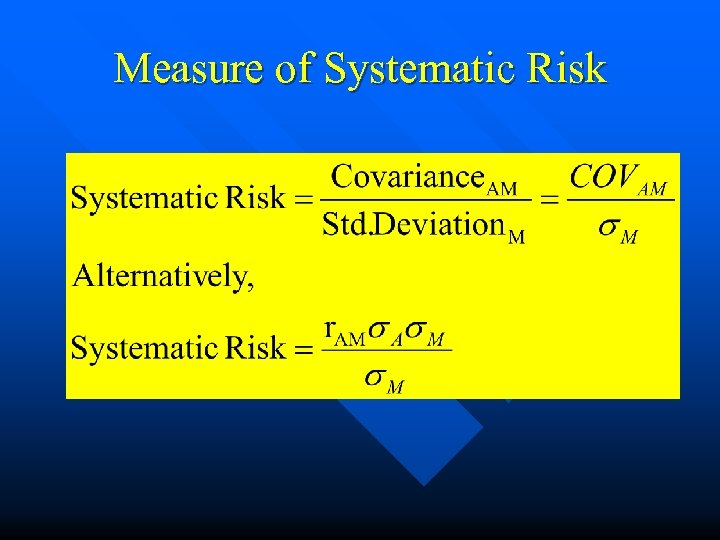Measure of Systematic Risk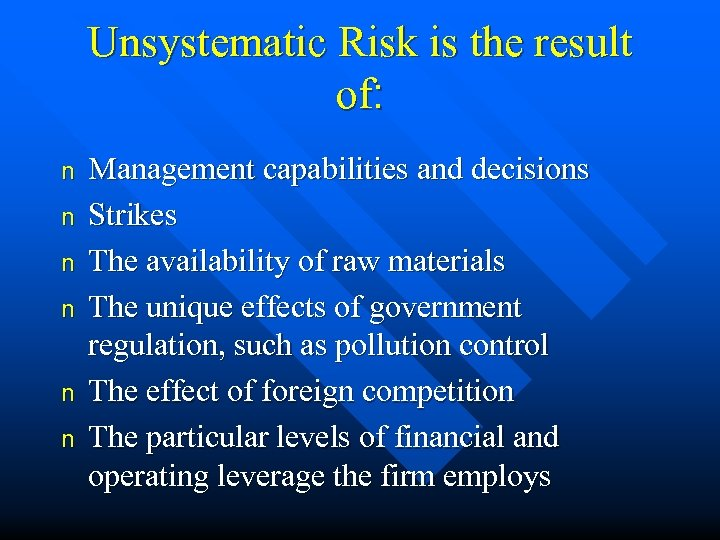Unsystematic Risk is the result of: n n n Management capabilities and decisions Strikes The availability of raw materials The unique effects of government regulation, such as pollution control The effect of foreign competition The particular levels of financial and operating leverage the firm employs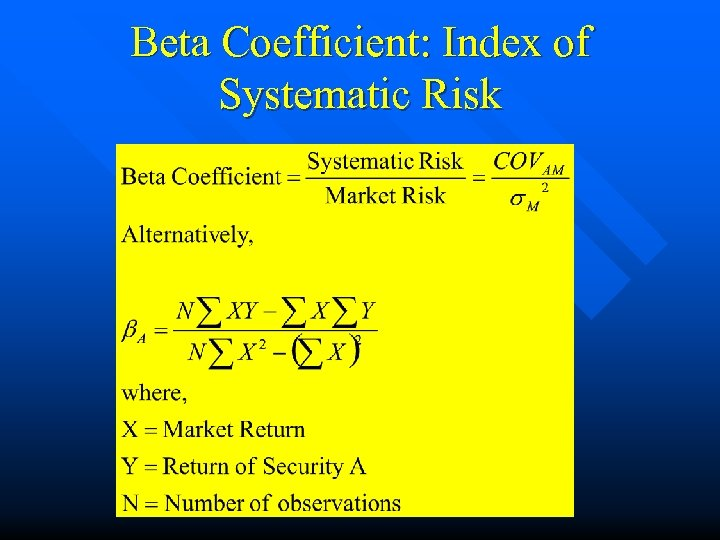Beta Coefficient: Index of Systematic Risk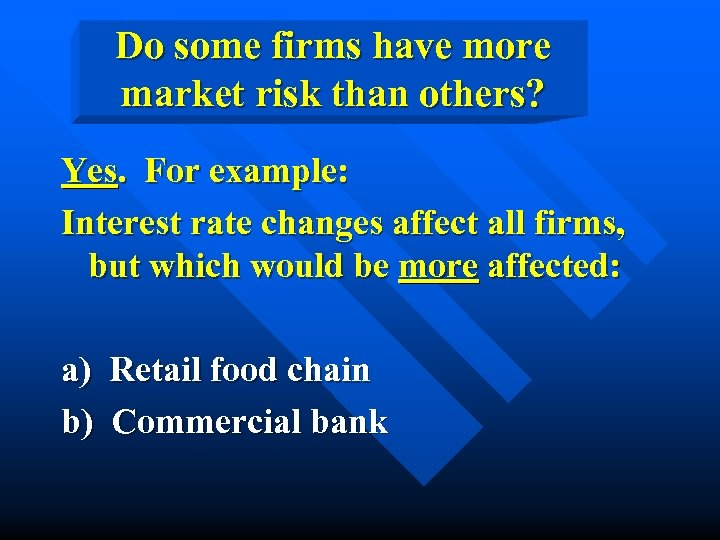Do some firms have more market risk than others? Yes. For example: Interest rate changes affect all firms, but which would be more affected: a) Retail food chain b) Commercial bank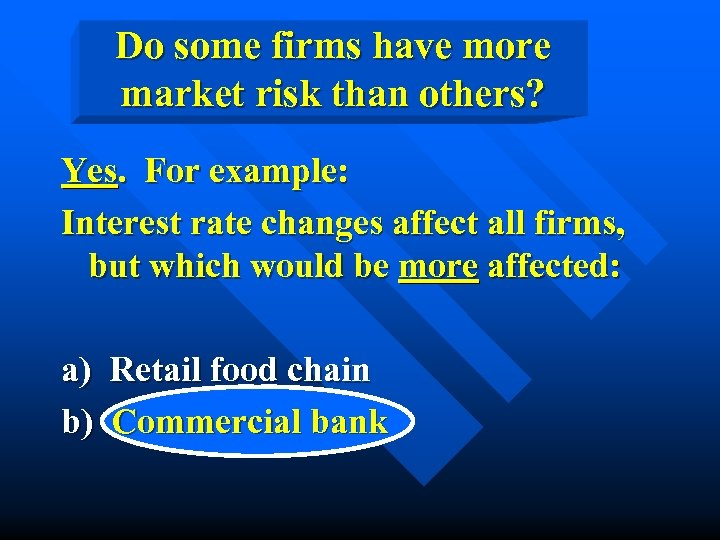Do some firms have more market risk than others? Yes. For example: Interest rate changes affect all firms, but which would be more affected: a) Retail food chain b) Commercial bank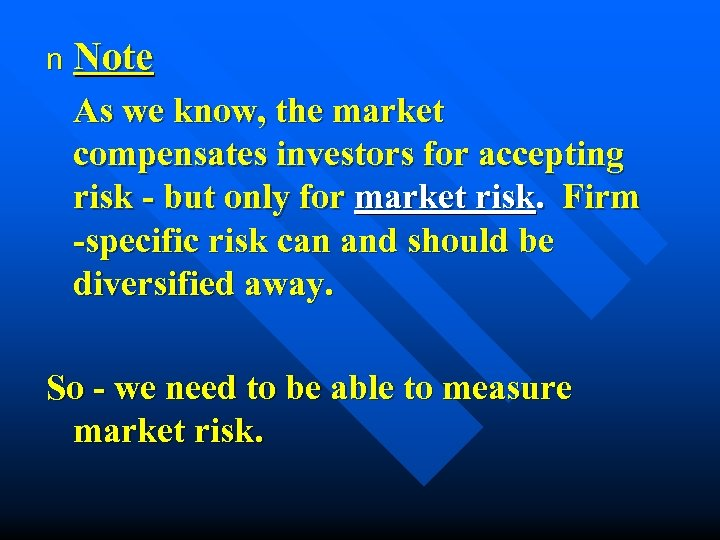n Note As we know, the market compensates investors for accepting risk - but only for market risk. Firm -specific risk can and should be diversified away. So - we need to be able to measure market risk.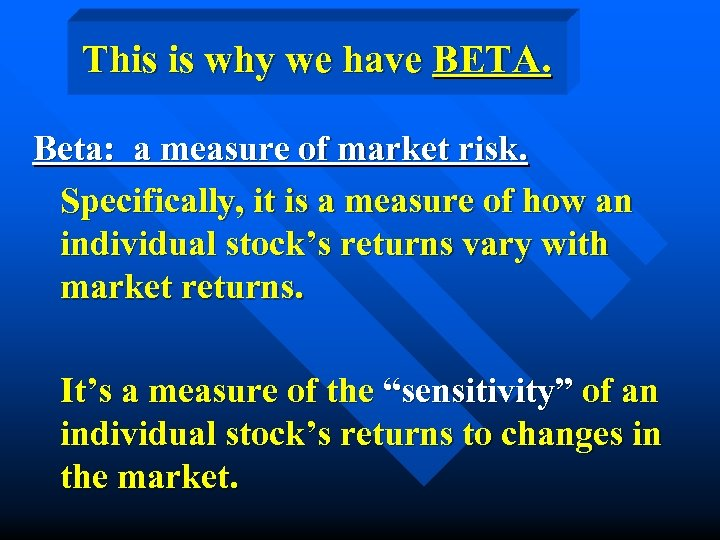This is why we have BETA. Beta: a measure of market risk. Specifically, it is a measure of how an individual stock’s returns vary with market returns. It’s a measure of the “sensitivity” of an individual stock’s returns to changes in the market.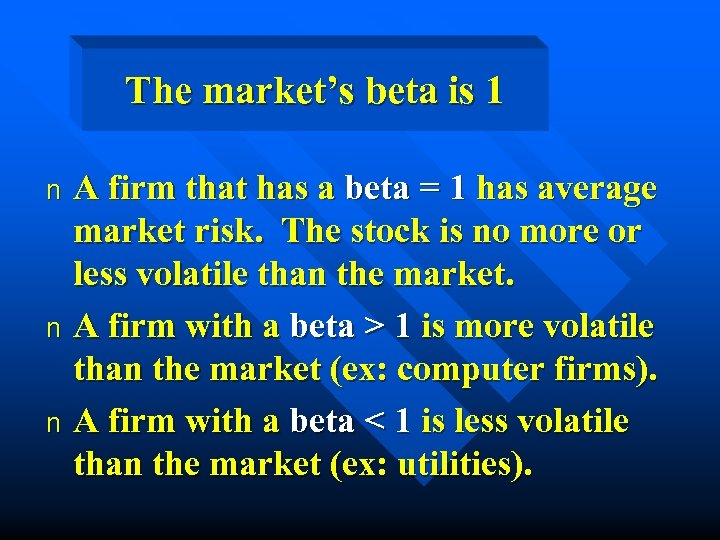The market’s beta is 1 A firm that has a beta = 1 has average market risk. The stock is no more or less volatile than the market. n A firm with a beta > 1 is more volatile than the market (ex: computer firms). n A firm with a beta < 1 is less volatile than the market (ex: utilities). n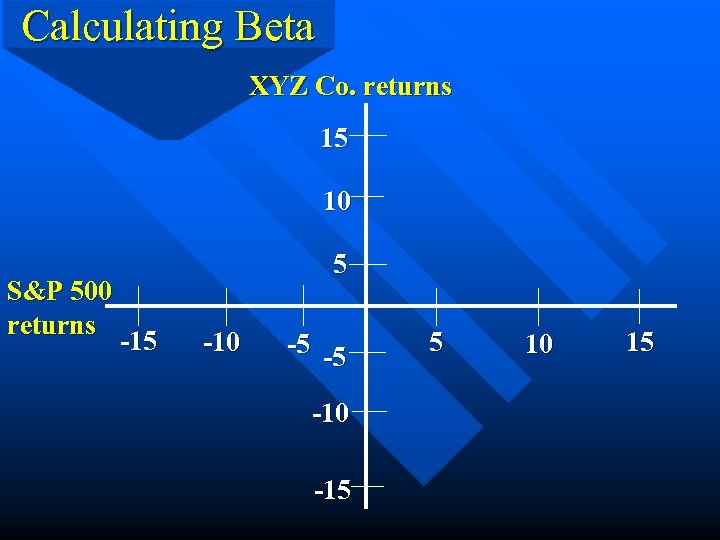Calculating Beta XYZ Co. returns 15 10 S&P 500 returns 5 -10 -5 -5 -10 -15 5 10 15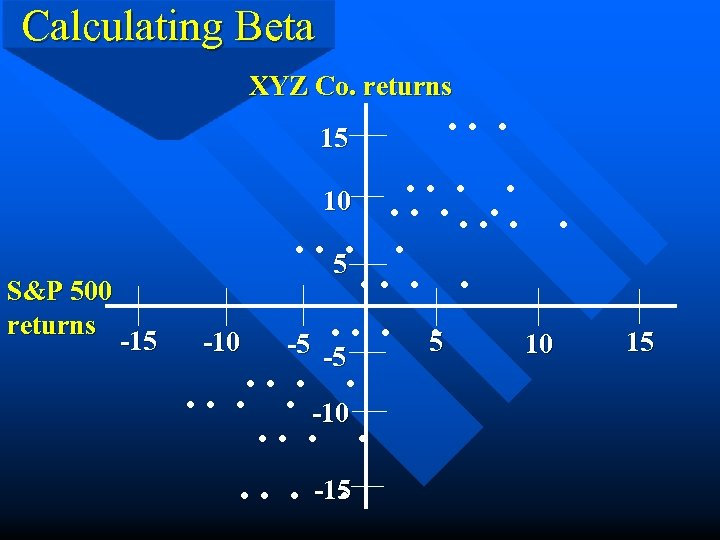Calculating Beta XYZ Co. returns 15 S&P 500 returns -15 . . . . 10. . . 5. -10 -5 -5 10. . . . -10. . . . -15. 15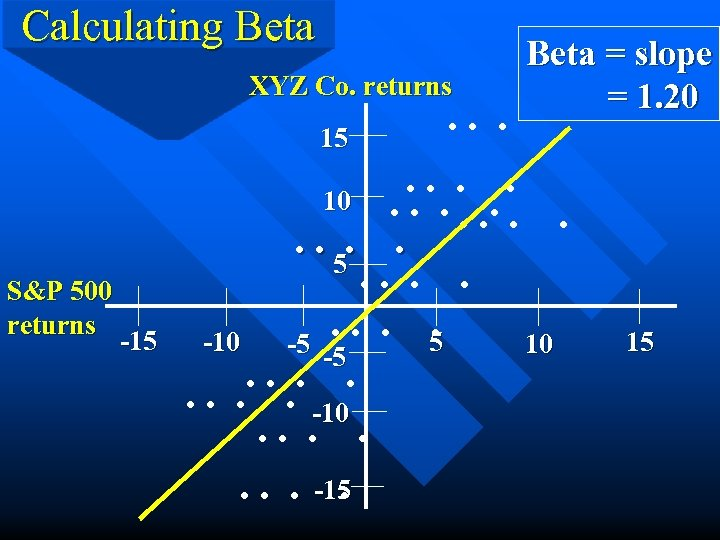Calculating Beta XYZ Co. returns 15 S&P 500 returns -15 . . . Beta = slope = 1. 20 . . 10. . . 5. -10 -5 -5 10. . . . -10. . . . -15. 15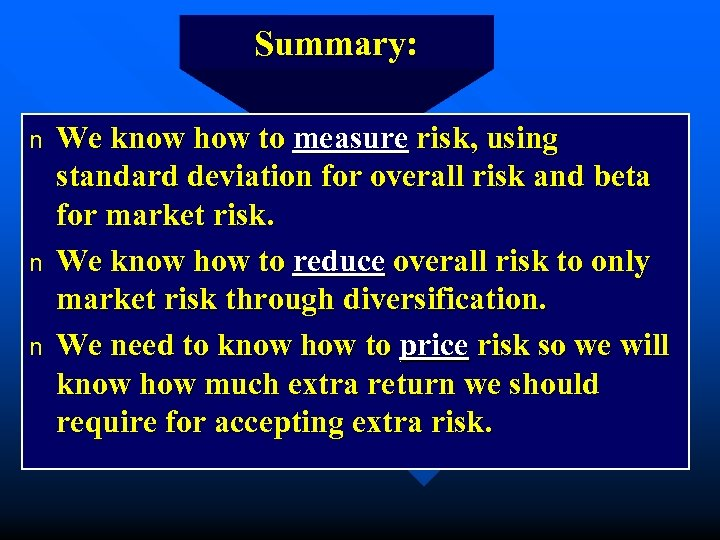Summary: n n n We know how to measure risk, using standard deviation for overall risk and beta for market risk. We know how to reduce overall risk to only market risk through diversification. We need to know how to price risk so we will know how much extra return we should require for accepting extra risk.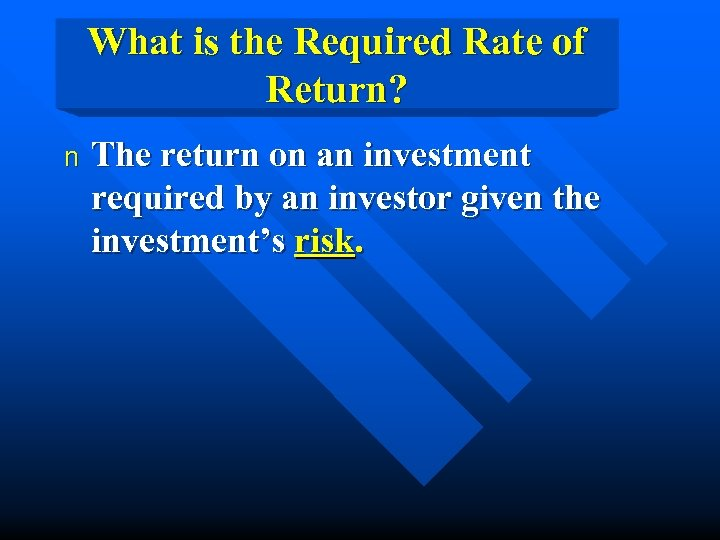What is the Required Rate of Return? n The return on an investment required by an investor given the investment’s risk.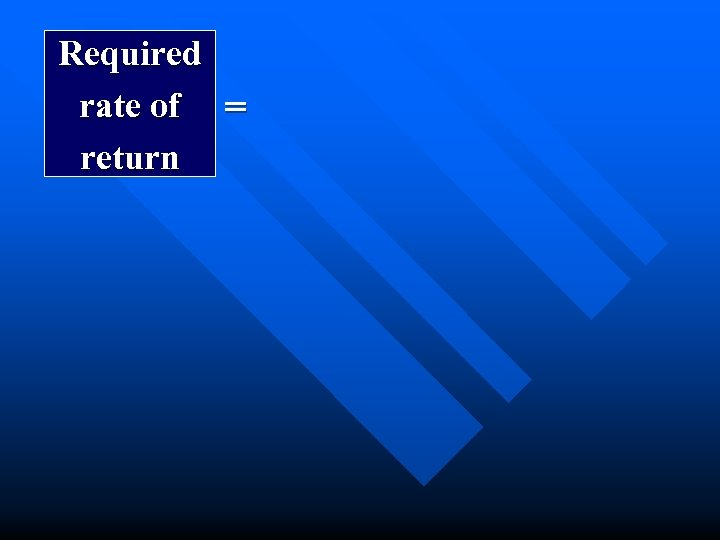Required rate of = return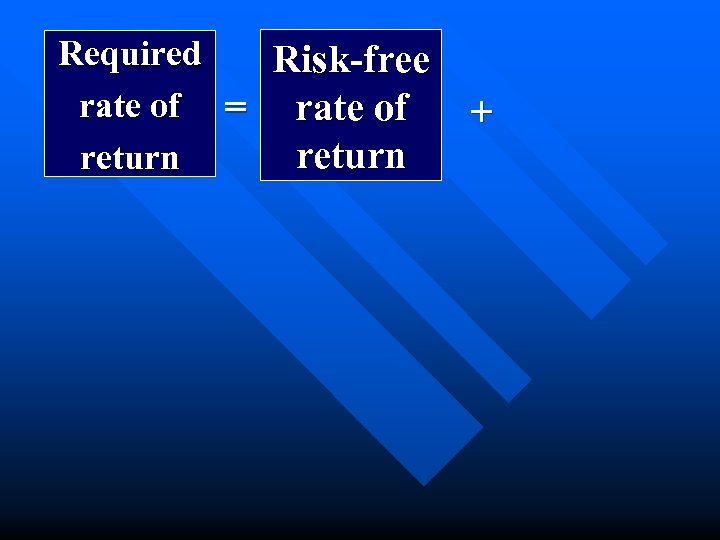Required Risk-free rate of = rate of return +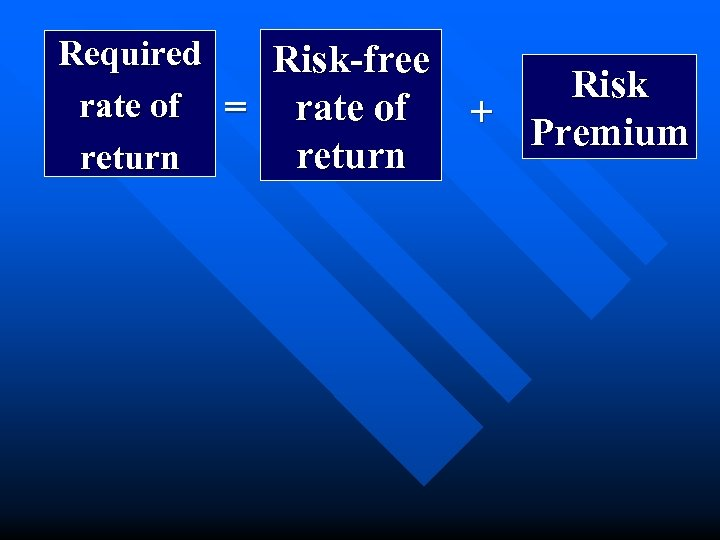Required Risk-free rate of = rate of return Risk + Premium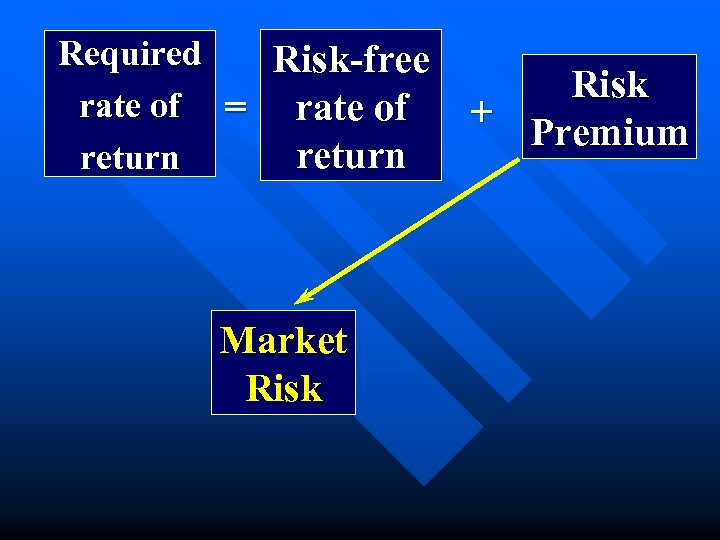Required Risk-free rate of = rate of return Market Risk + Premium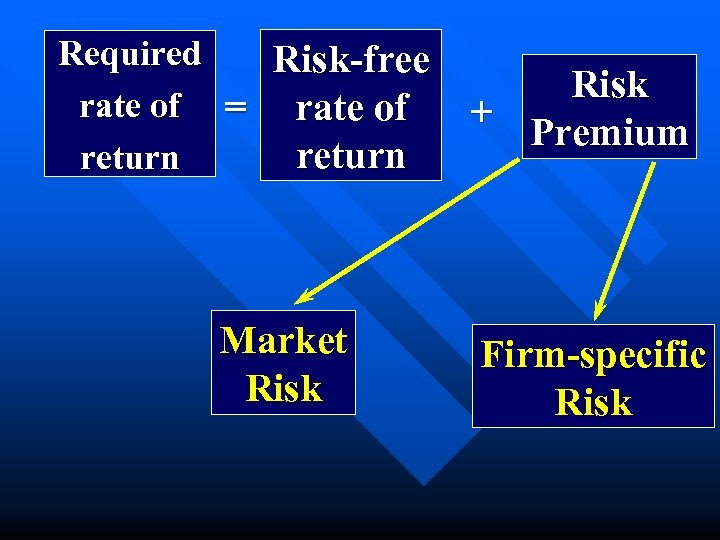Required Risk-free rate of = rate of return Market Risk + Premium Firm-specific Risk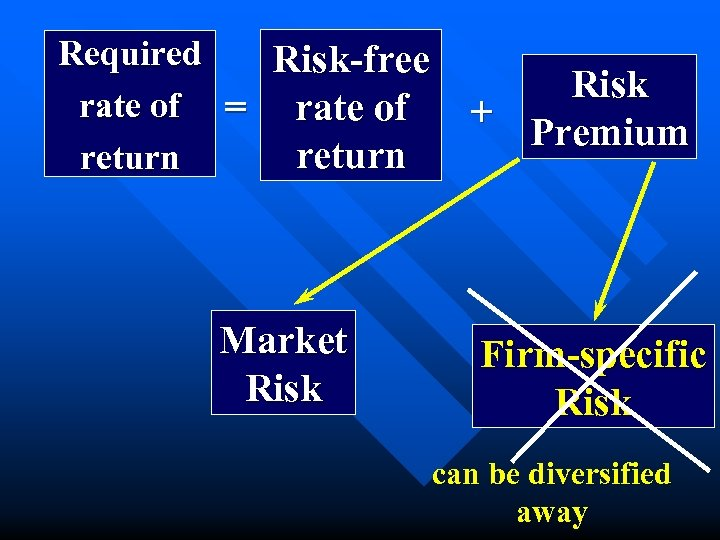Required Risk-free rate of = rate of return Market Risk + Premium Firm-specific Risk can be diversified away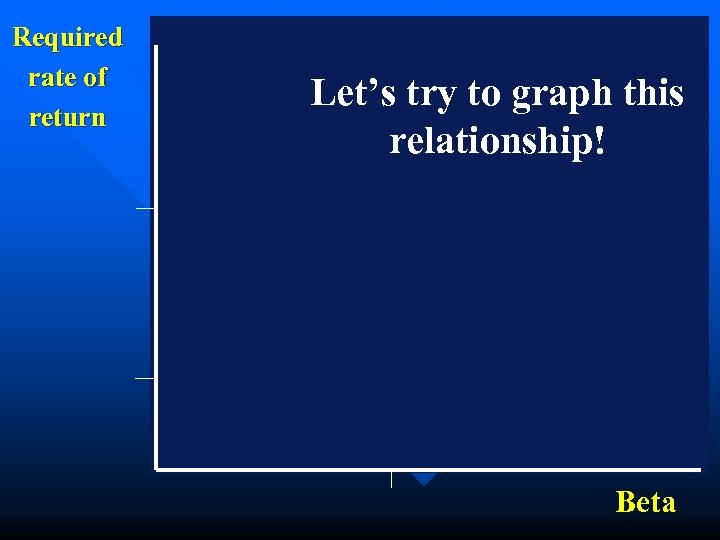Required rate of return Let’s try to graph this relationship! Beta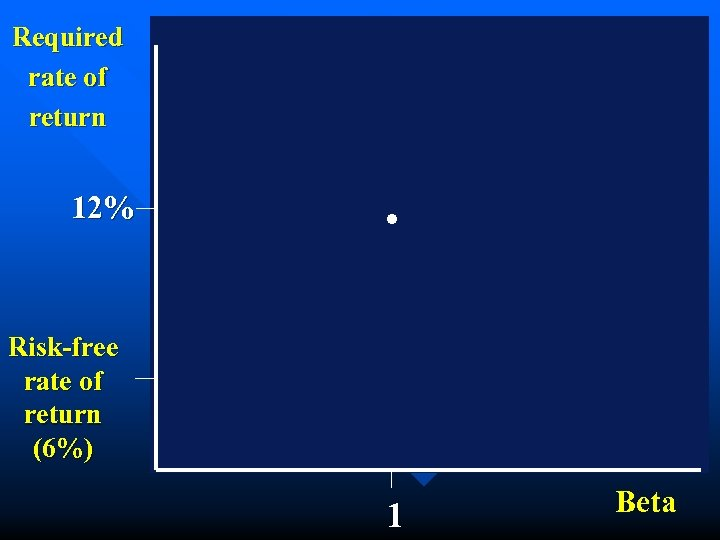Required rate of return 12% . Risk-free rate of return (6%) 1 Beta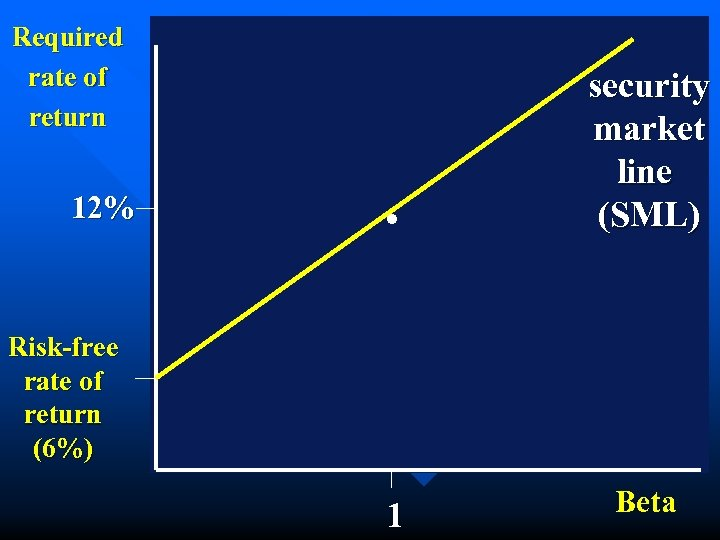Required rate of return 12% . security market line (SML) Risk-free rate of return (6%) 1 Beta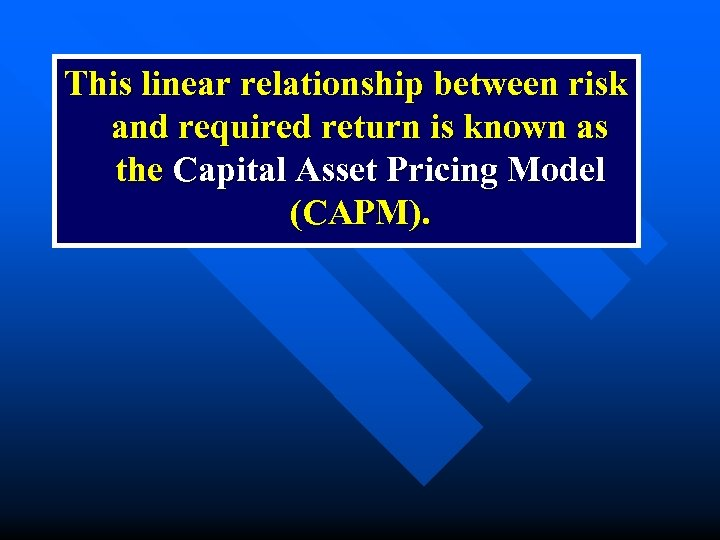This linear relationship between risk and required return is known as the Capital Asset Pricing Model (CAPM).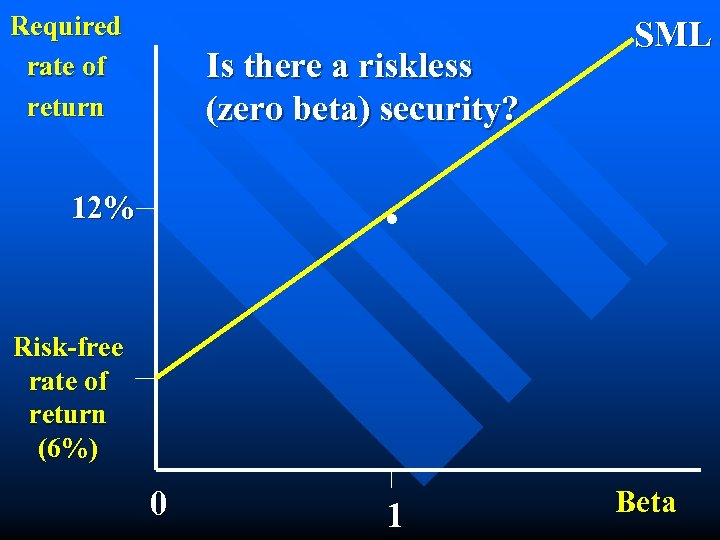Required rate of return Is there a riskless (zero beta) security? SML . 12% Risk-free rate of return (6%) 0 1 Beta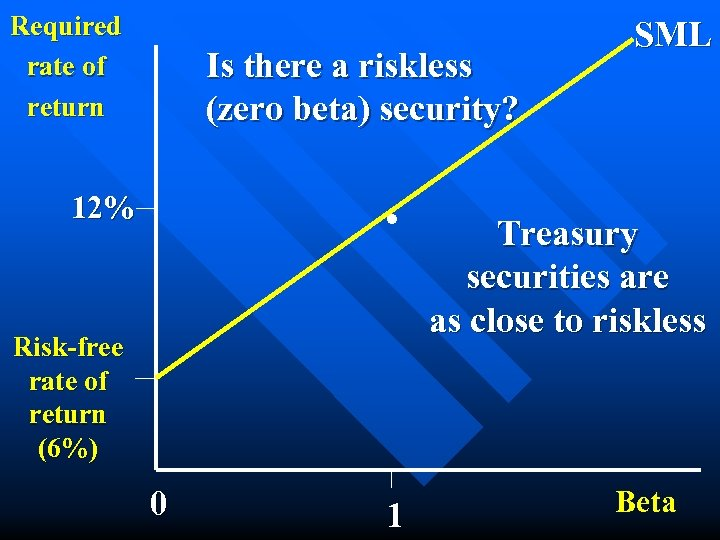Required rate of return Is there a riskless (zero beta) security? . 12% Risk-free rate of return (6%) 0 1 SML Treasury securities are as close to riskless Beta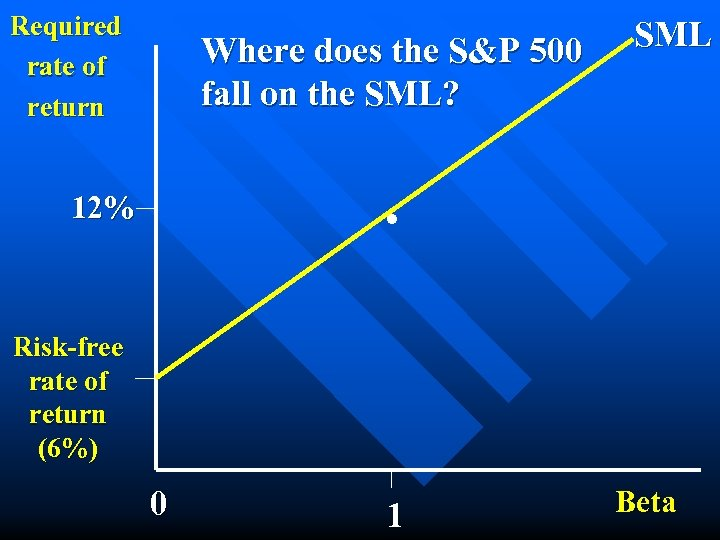Required rate of return Where does the S&P 500 fall on the SML? SML . 12% Risk-free rate of return (6%) 0 1 Beta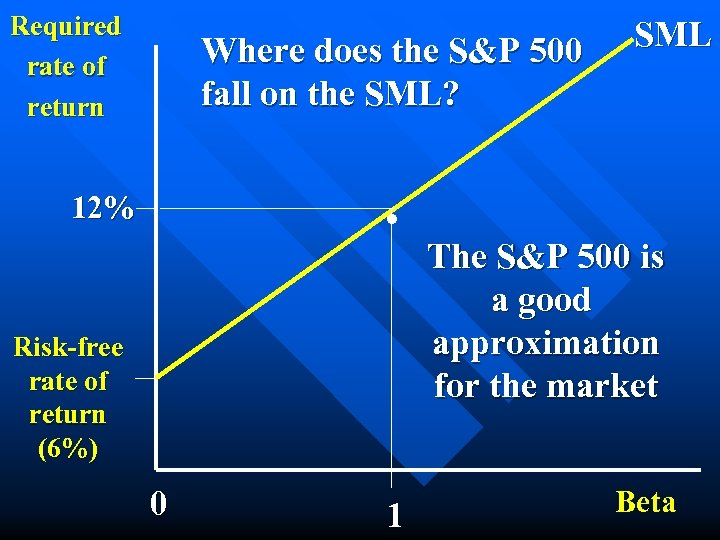Required rate of return Where does the S&P 500 fall on the SML? . 12% Risk-free rate of return (6%) 0 1 SML The S&P 500 is a good approximation for the market Beta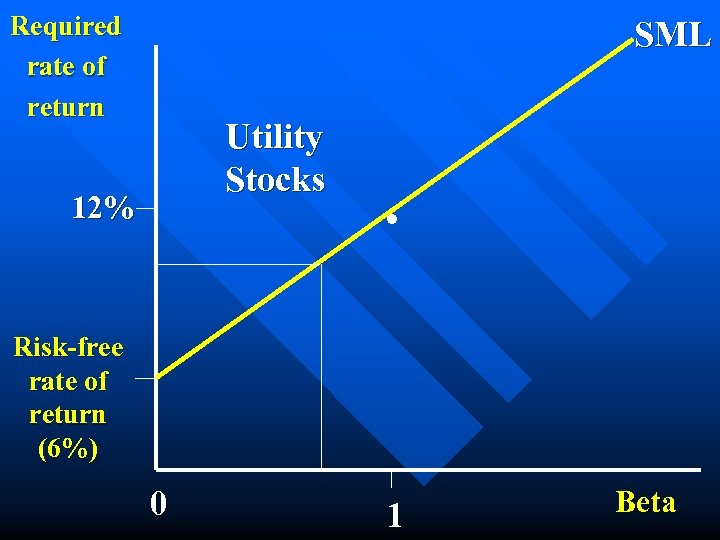Required rate of return SML Utility Stocks 12% . Risk-free rate of return (6%) 0 1 Beta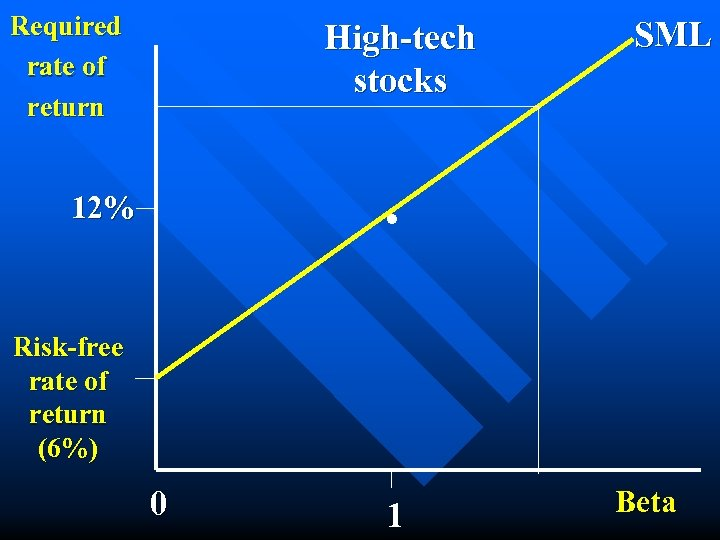Required rate of return High-tech stocks SML . 12% Risk-free rate of return (6%) 0 1 Beta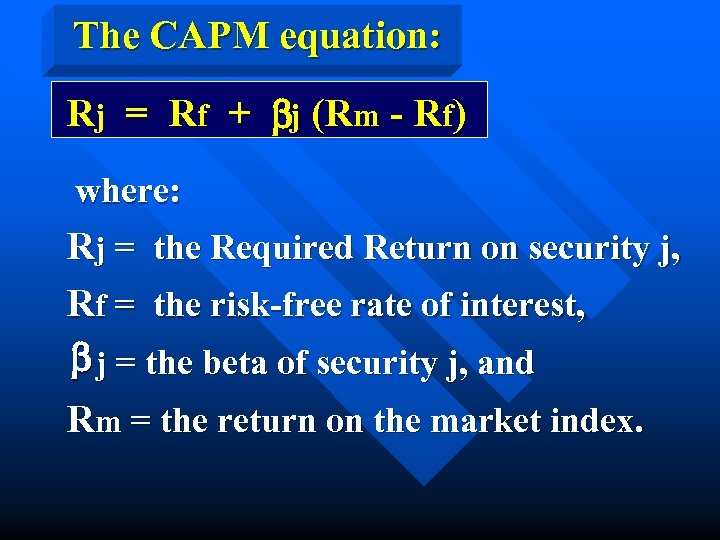The CAPM equation: Rj = Rf + bj (Rm - Rf) where: Rj = the Required Return on security j, Rf = the risk-free rate of interest, b j = the beta of security j, and Rm = the return on the market index.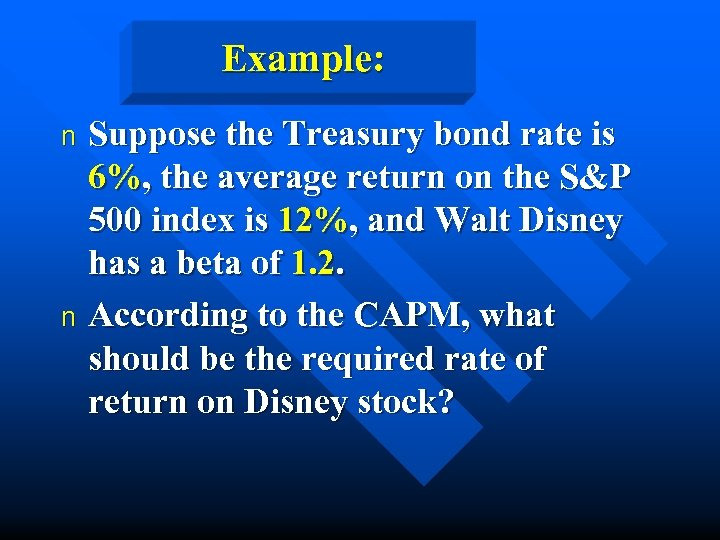Example: Suppose the Treasury bond rate is 6%, the average return on the S&P 500 index is 12%, and Walt Disney has a beta of 1. 2. n According to the CAPM, what should be the required rate of return on Disney stock? n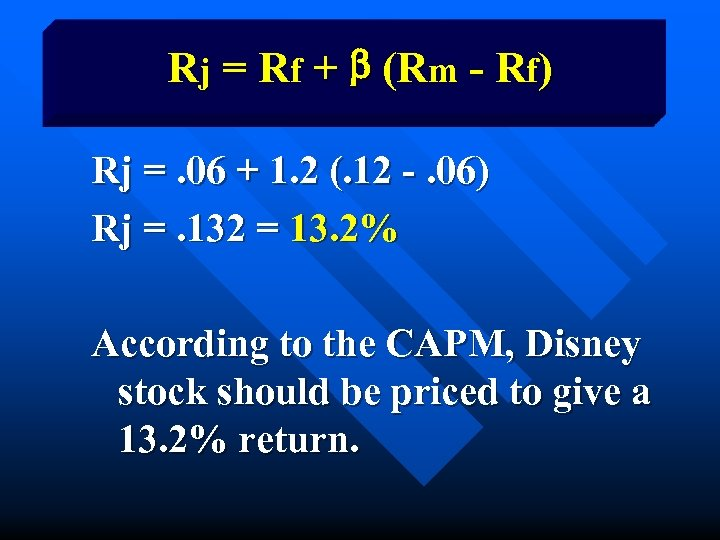Rj = Rf + b (Rm - Rf) Rj =. 06 + 1. 2 (. 12 -. 06) Rj =. 132 = 13. 2% According to the CAPM, Disney stock should be priced to give a 13. 2% return.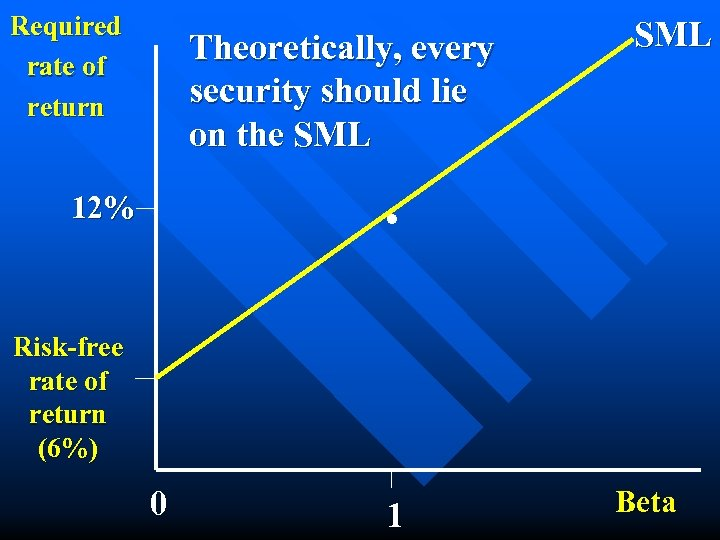Required rate of return Theoretically, every security should lie on the SML . 12% Risk-free rate of return (6%) 0 1 Beta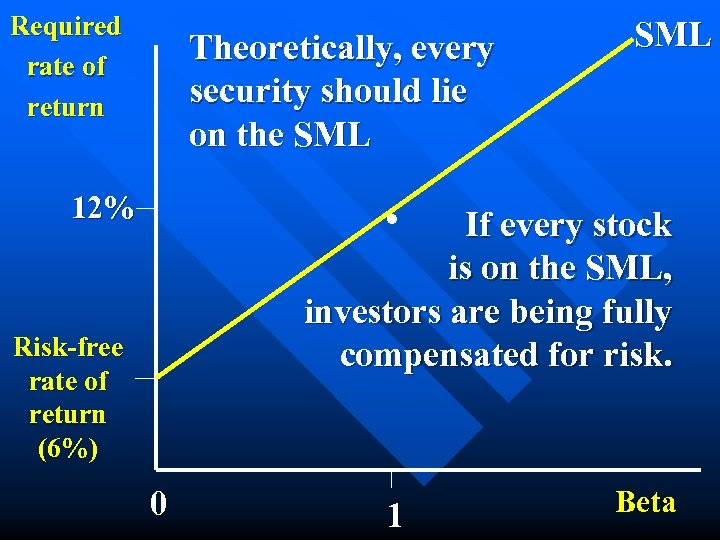Required rate of return Theoretically, every security should lie on the SML . 12% If every stock is on the SML, investors are being fully compensated for risk. Risk-free rate of return (6%) 0 1 Beta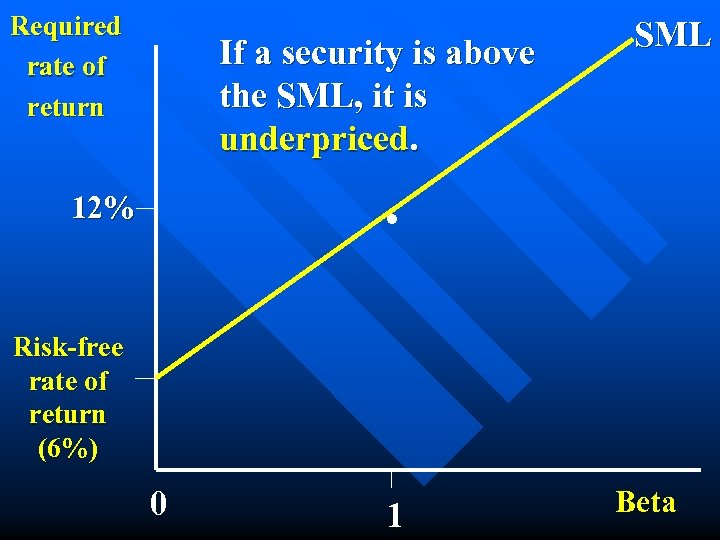Required rate of return If a security is above the SML, it is underpriced. SML . 12% Risk-free rate of return (6%) 0 1 Beta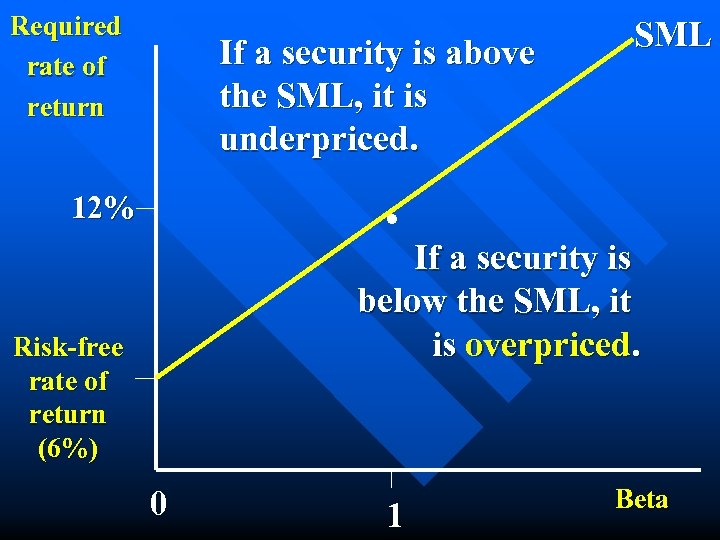Required rate of return If a security is above the SML, it is underpriced. SML . 12% If a security is below the SML, it is overpriced. Risk-free rate of return (6%) 0 1 Beta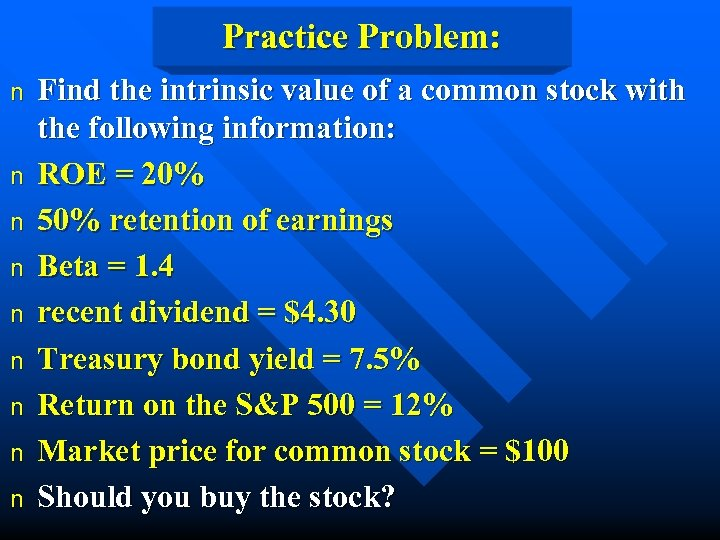Practice Problem: n n n n n Find the intrinsic value of a common stock with the following information: ROE = 20% 50% retention of earnings Beta = 1. 4 recent dividend = \$4. 30 Treasury bond yield = 7. 5% Return on the S&P 500 = 12% Market price for common stock = \$100 Should you buy the stock?